• Archive by category "程序算法"

# Blog Archives

## 用R语言10分钟上手神经网络模型

R的极客理想系列文章，涵盖了R的思想，使用，工具，创新等的一系列要点，以我个人的学习和体验去诠释R的强大。

R语言作为统计学一门语言，一直在小众领域闪耀着光芒。直到大数据的爆发，R语言变成了一门炙手可热的数据分析的利器。随着越来越多的工程背景的人的加入，R语言的社区在迅速扩大成长。现在已不仅仅是统计领域，教育，银行，电商，互联网….都在使用R语言。

• 张丹，分析师/程序员/Quant: R,Java,Nodejs
• blog: http://blog.fens.me
• email: bsspirit@gmail.com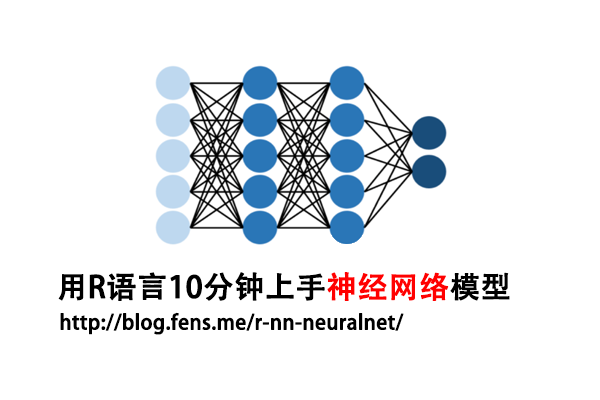“神经网络”，“深度学习”，已经不是纯学术的词了，有很多的算法已经落地为我们生活中的各种产品，比如人脸识别，智能客服，从图片中提词，AI作诗，AI作画，chatGPT，AphlaGo等，各种的AI算法应用出圈，打破很多行业壁垒，甚至机器比人类更有创造力，做的更好。这些AI应用出圈的背后，很大程度上是依赖于神经网络的高速发展。

1. 神经网络介绍
2. neuralnet包介绍
3. 神经元模型：零隐藏层
4. 单层神经网络（感知机）：一个隐藏层
5. 单层神经网络：多分类
6. 两层神经网络（多层感知器）：多分类
7. 多层神经网络（深度学习）

## 1. 神经网络介绍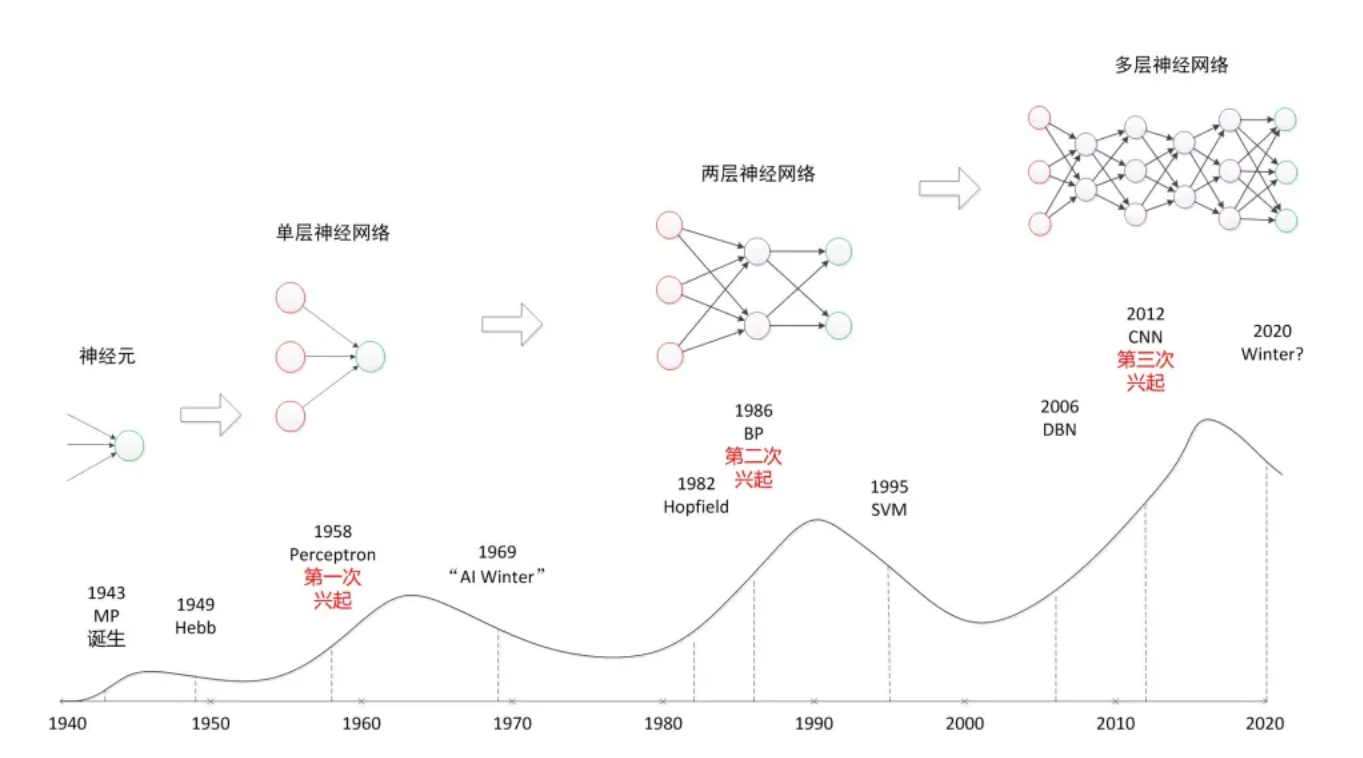• 输入节点：接受与处理训练数据集中的各输入变量值
• 隐藏点：实现非线性数据的线性变换
• 输出节点：给出输出变量的分类或预测结果

## 2. neuralnet包介绍

neuralnet包，安装过程很简单。

``````
# 安装
> install.packages("neuralnet")

# 加载
> library(neuralnet)

# 设置工作路径
> setwd("C:/work/R/neural/neural_networks")
``````

neuralnet包中的，neuralnet()函数可用于神经网络的模型训练，是这个包中最核心的函数，neuralnet()函数参数很多，是需要我们仔细了解的。

neuralnet()函数，在训练神经网络模型时，支持使用反向传播(BP)、弹性反向传播（RPROP）、无权重回溯、修正的全球收敛版本（GRPROP）训练神经网络，函数允许通过自定义选择误差和激活函数进行灵活设置。此外，还实现了广义权重的计算。

``````
> neuralnet
function (formula, data, hidden = 1, threshold = 0.01, stepmax = 1e+05,
rep = 1, startweights = NULL, learningrate.limit = NULL,
learningrate.factor = list(minus = 0.5, plus = 1.2), learningrate = NULL,
lifesign = "none", lifesign.step = 1000, algorithm = "rprop+",
err.fct = "sse", act.fct = "logistic", linear.output = TRUE,
exclude = NULL, constant.weights = NULL, likelihood = FALSE)

...
``````

• formula: 定义模型的公式。
• data: data.frame格式，训练集原始数据。
• hidden: 整数向量，指定每层中隐藏神经元的数量，如c(3,2)为二个隐藏层，第一层3个节点，第二层2个节点。
• threshold: 数值型，停止条件阈值，指定误差函数的偏导数作为停止标准的阈值。
• stepmax: 数值型，停止条件最大迭代次数，达到这个最大值就会停止神经网络的训练过程。
• rep: 神经网络训练的重复次数。
• startweights: 模型起始值的权重向量，设置为NULL用于随机初始化。
• learningrate.limit: 一个向量或一个列表，包含学习率的最低和最高限制，仅用于RPROP和GRPROP。
• learningrate.factor: 一个向量或一个列表，包含学习率的上限和下限的乘法系数，仅用于RPROP和GRPROP。
• learningrate: 数值型，指定传统反向传播使用的学习率，只用于传统的反向传播。
• lifesign: 字符串，指定函数在计算神经网络时的打印量，’none’, ‘minimal’ or ‘full’。
• lifesign.step: 整数型，指定在完全lifesign模式下打印最小阈值的步长。
• algorithm: 字符串，包含用于计算神经网络的算法类型。以下类型是可能的：”backprop”，”rprop+”，”rprop-“，”sag”，或 “slr”。backprop’指的是反向传播，’rprop+’和’rprop-‘指的是带有和不带有权重回溯的弹性反向传播，而’sag’和’slr’则是诱导使用修改后的全局收敛算法（GRPROP）。
• err.fct: 损失函数，用于计算误差的函数，可以使用字符串’sse’和’ce’，它们代表平方误差之和和交叉熵。
• act.fct: 激活函数，用于平滑协变量或神经元与权重的交叉积的结果。字符串 “logistic”和 “tanh” 可以用于logistic函数和切线双曲。
• linear.output：逻辑值，是否线性输出，即是回归还是分类。TRUE表示输出节点的激活函数为线性函数,FALSE表示非线性函数,在B-P中为FALSE
• exclude: 一个向量或一个矩阵，指定从计算中排除的权重。如果给定的是一个向量，必须知道权重的确切位置。一个有n行3列的矩阵将排除n个权重，其中第一列代表层，第二列代表输入神经元，第三列代表权重的输出神经元。
• constant.weights: 一个向量，指定训练过程中不需要训练的权重，固定值。
• likelihood: 逻辑值，如果误差函数等于负对数似然函数，将计算信息准则AIC和BIC。

## 3. 神经元模型：零隐藏层

``````
Sepal.Length Sepal.Width Petal.Length Petal.Width Species
1          5.1         3.5          1.4         0.2  setosa
2          4.9         3.0          1.4         0.2  setosa
3          4.7         3.2          1.3         0.2  setosa
4          4.6         3.1          1.5         0.2  setosa
5          5.0         3.6          1.4         0.2  setosa
6          5.4         3.9          1.7         0.4  setosa
``````

``````
# 没有隐藏层
> nn <- neuralnet(Species=="setosa"~ Sepal.Length + Sepal.Width + Petal.Length + Petal.Width,
+                 data = iris,hidden = 0)

# 查看网络结构
> plot(nn)
``````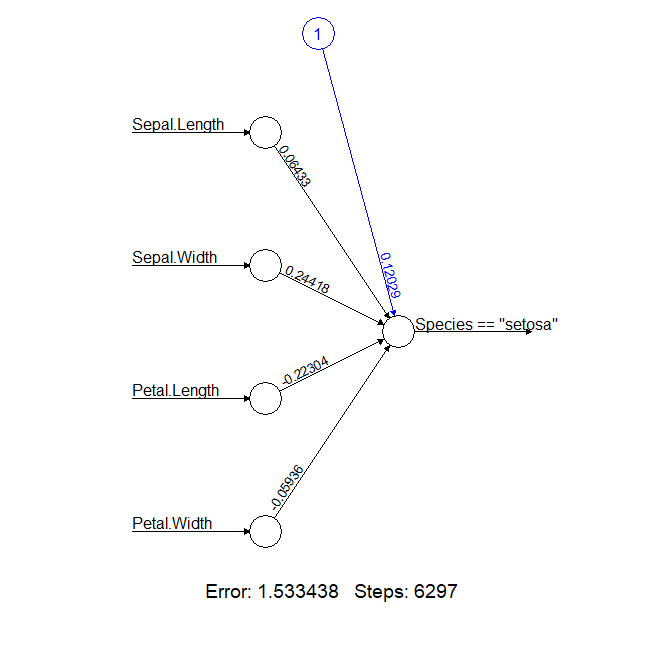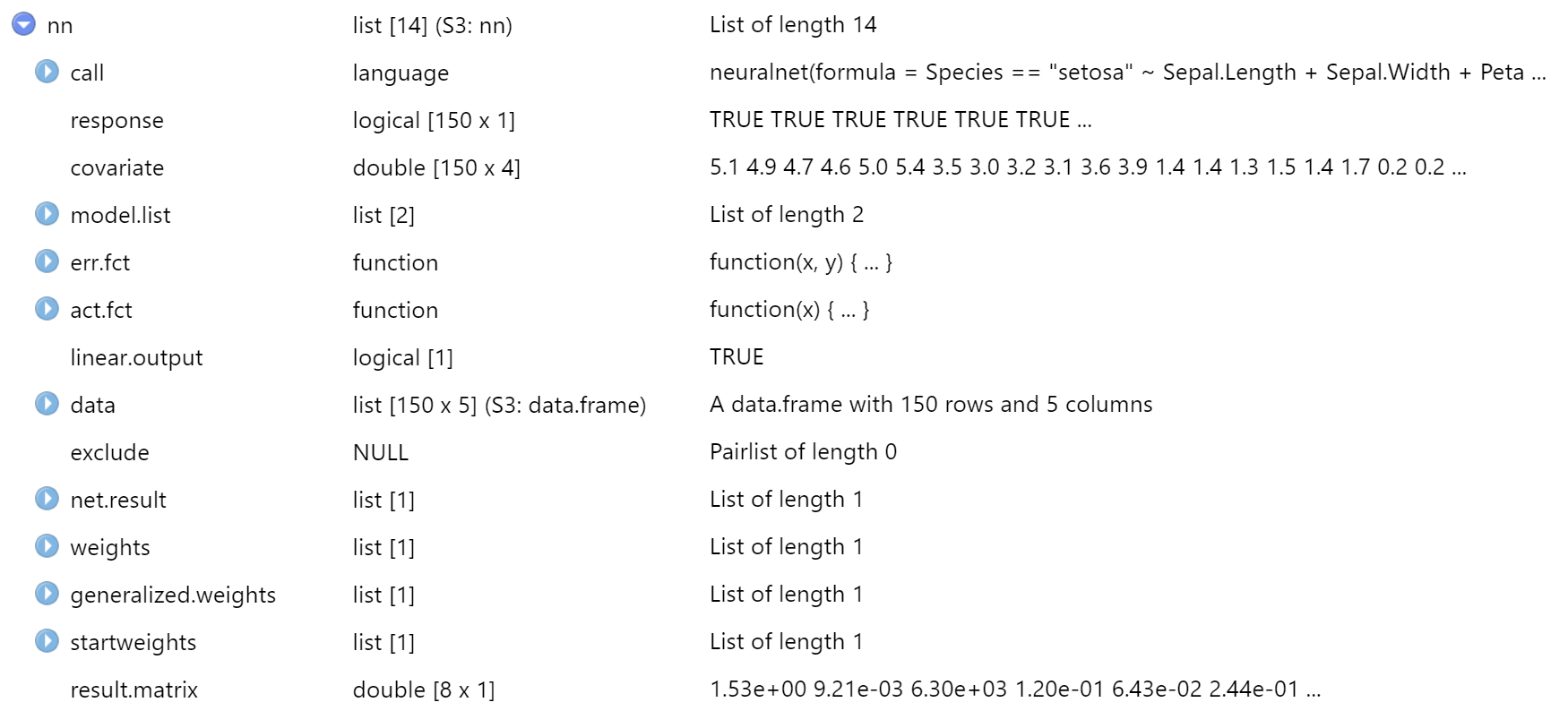• call：模型函数
• response：因变量数据
• covariate：自变量数据
• model.list：模型函数
• err.fct：损失函数
• act.fct：激活函数
• linear.output：是否线性输出
• data：原始数据集
• exclude：指定从计算中排除的权重
• net.result：预测值结果
• weights：各个节点的权重值列表
• generalized.weights：广义权重
• startweights：各个节点的初始权重（初始权值为在的正态分布随机数）
• result.matrix：结果矩阵，终止迭代时各个节点的权重，迭代次数，损失函数值和权重的最大调整量

``````> nn\$result.matrix
[,1]
error                                1.533438e+00
reached.threshold                    9.212915e-03
steps                                6.297000e+03
Intercept.to.Species == "setosa"     1.202943e-01
Sepal.Length.to.Species == "setosa"  6.432600e-02
Sepal.Width.to.Species == "setosa"   2.441786e-01
Petal.Length.to.Species == "setosa" -2.230368e-01
Petal.Width.to.Species == "setosa"  -5.935682e-02
``````
• error，损失函数值，1.533438，值越小
• reached.threshold，终止条件，权重的最大调整量9.212915e-03
• steps，整个训练执行了6297步
• Intercept.to.Species，偏差权重（截距），值为1.202943e-01
• Sepal.Length.to.Species，Sepal.Length变量权重
• Sepal.Width.to.Species，Sepal.Width变量权重
• Petal.Length.to.Species，Petal.Length变量权重
• Petal.Width.to.Species，Petal.Width变量权重

``````
> nn\$startweights
[]
[][]
[,1]
[1,]  0.5797599
[2,] -0.3526484
[3,]  1.7074446
[4,]  1.3395568
[5,]  0.7781903
``````

``````
> nn\$weights
[]
[][]
[,1]
[1,]  0.12029435
[2,]  0.06432600
[3,]  0.24417858
[4,] -0.22303683
[5,] -0.05935682
``````

``````
# 取前6条
[,1]       [,2]       [,3]        [,4]
[1,]  3.1084343  11.799476 -10.777840  -2.8683080
[2,]  0.4883183   1.853634  -1.693141  -0.4505957
[3,]  0.7288879   2.766825  -2.527265  -0.6725814
[4,]  0.4490363   1.704522  -1.556939  -0.4143483
[5,] 20.4485892  77.621919 -70.901170 -18.8689374
[6,] -3.7104548 -14.084718  12.865219   3.4238224
``````

``````
# 模型公式
> nn\$call
neuralnet(formula = Species == "setosa" ~ Sepal.Length + Sepal.Width +
Petal.Length + Petal.Width, data = iris, hidden = 0)

# 损失函数
> nn\$err.fct
function (x, y)
{
1/2 * (y - x)^2
}

attr(,"type")
 "sse"

# 激活函数
> nn\$act.fct
function (x)
{
1/(1 + exp(-x))
}

attr(,"type")
 "logistic"
``````

``````
# 模型的变量定义
> nn\$model.list
\$response
 "Species == \"setosa\""

\$variables
 "Sepal.Length" "Sepal.Width"  "Petal.Length" "Petal.Width"

# 查看自变量，取前6条
Sepal.Length Sepal.Width Petal.Length Petal.Width
[1,]          5.1         3.5          1.4         0.2
[2,]          4.9         3.0          1.4         0.2
[3,]          4.7         3.2          1.3         0.2
[4,]          4.6         3.1          1.5         0.2
[5,]          5.0         3.6          1.4         0.2
[6,]          5.4         3.9          1.7         0.4
``````

``````
# 查看因变量，实际值，取前6条
Species == "setosa"
1                TRUE
2                TRUE
3                TRUE
4                TRUE
5                TRUE
6                TRUE

# 模型预测结果，取前6条
[,1]
[1,] 0.9788590
[2,] 0.8439046
[3,] 0.9021788
[4,] 0.8267209
[5,] 0.9968443
[6,] 1.0170459
``````

## 4. 单层神经网络（感知机）：一个隐藏层

``````
# 训练
> n1 <- neuralnet(Species=="setosa"~ Sepal.Length + Sepal.Width + Petal.Length + Petal.Width,
+ data = iris,hidden=1)

# 画出网络结构
> plot(n1)
``````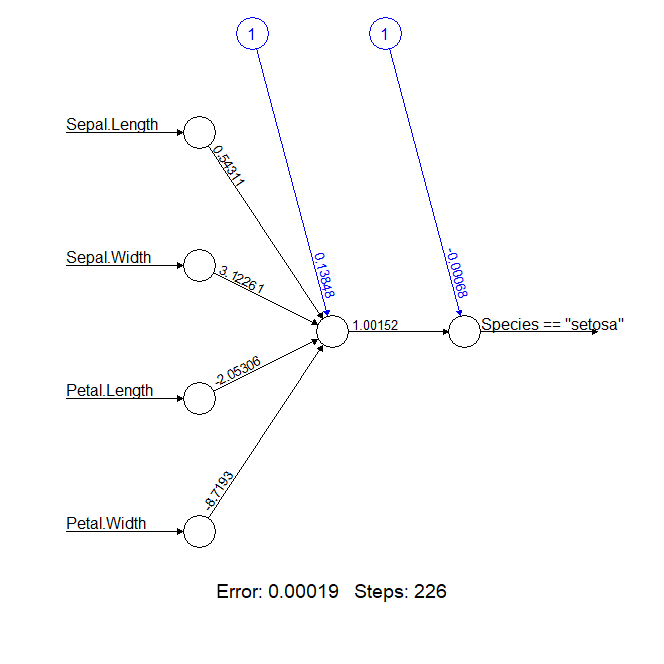``````
> n1\$result.matrix
[,1]
error                             1.899890e-04
reached.threshold                 9.246323e-03
steps                             2.260000e+02
Intercept.to.1layhid1             1.384797e-01
Sepal.Length.to.1layhid1          5.431059e-01
Sepal.Width.to.1layhid1           3.122608e+00
Petal.Length.to.1layhid1         -2.053056e+00
Petal.Width.to.1layhid1          -8.719302e+00
Intercept.to.Species == "setosa" -6.759521e-04
1layhid1.to.Species == "setosa"   1.001522e+00
``````

error的损失值为0.00019，整个训练执行了226步，比神经元模型的零隐藏层，有了大幅的提升。一下子就把分类问题解决了，太神奇了吧。

## 5. 单层神经网络：多分类

``````
# 单层网络
> n2a <- neuralnet(Species~ Sepal.Length + Sepal.Width + Petal.Length + Petal.Width,
+ data = iris,hidden=1)

# 画图
> plot(n2a)
``````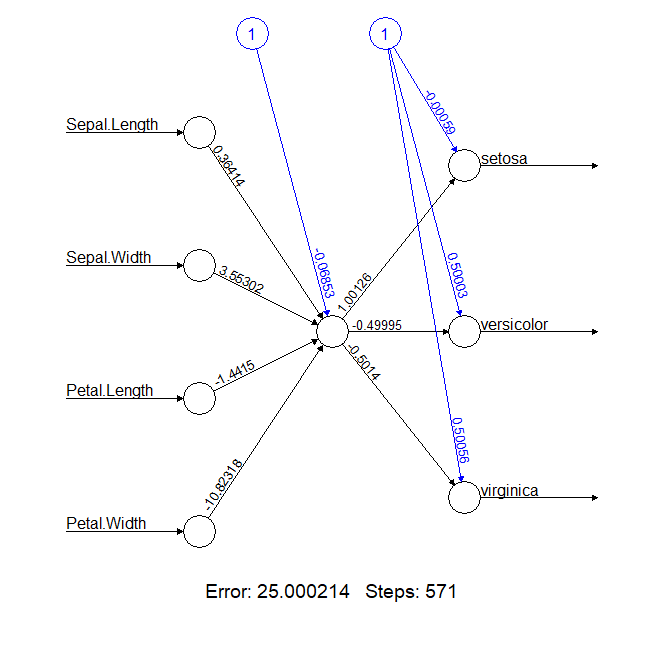``````
# 单层网络，2个节点
> n2b <- neuralnet(Species~ Sepal.Length + Sepal.Width + Petal.Length + Petal.Width,
+ data = iris,hidden=c(2))

> plot(n2b)
``````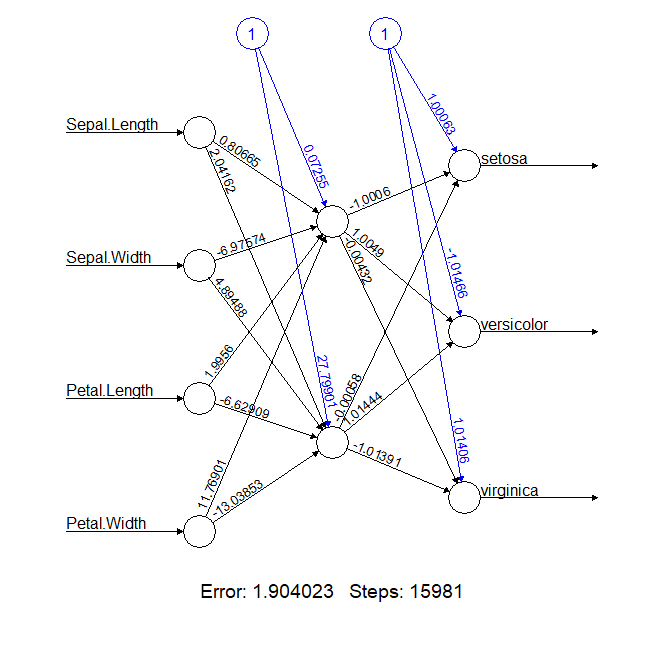## 6. 两层神经网络（多层感知器）：多分类

``````
# 2个隐藏层，每层1个节点
> n3a <- neuralnet(Species~ Sepal.Length + Sepal.Width + Petal.Length + Petal.Width, + data = iris,hidden=c(1,1)) > n3a\$result.matrix
[,1]
error                     25.000112366
reached.threshold          0.007309118
steps                    358.000000000
Intercept.to.1layhid1      2.677698687
Sepal.Length.to.1layhid1  -0.737245035
Sepal.Width.to.1layhid1    3.844506938
Petal.Length.to.1layhid1  -1.018677154
Petal.Width.to.1layhid1  -10.512582993
Intercept.to.2layhid1     -0.771711595
1layhid1.to.2layhid1       3.469423693
Intercept.to.setosa       -0.510039484
2layhid1.to.setosa         1.612291660
Intercept.to.versicolor    0.754101331
2layhid1.to.versicolor    -0.804506879
Intercept.to.virginica     0.755525924
2layhid1.to.virginica     -0.807147653
``````

error达到了25，又是一个不可接受的结果。

``````
# 2个隐藏层，每层2个节点
> n3b <- neuralnet(Species~ Sepal.Length + Sepal.Width + Petal.Length + Petal.Width, + data = iris,hidden=c(2,2)) # 结果矩阵 > n3b\$result.matrix
[,1]
error                    24.999473404
reached.threshold         0.008004802
steps                    83.000000000
Intercept.to.1layhid1    -0.493094700
Sepal.Length.to.1layhid1 -1.519304930
Sepal.Width.to.1layhid1  -1.217278013
Petal.Length.to.1layhid1  3.491582287
Petal.Width.to.1layhid1   3.469063900
Intercept.to.1layhid2    -0.921659140
Sepal.Length.to.1layhid2 -2.263419044
Sepal.Width.to.1layhid2   0.410851516
Petal.Length.to.1layhid2  1.581399076
Petal.Width.to.1layhid2  -1.453986728
Intercept.to.2layhid1     0.898644804
1layhid1.to.2layhid1     -2.635824185
1layhid2.to.2layhid1     -2.577338580
Intercept.to.2layhid2    -0.829242612
1layhid1.to.2layhid2     -0.706157301
1layhid2.to.2layhid2      1.549670915
Intercept.to.setosa      -0.436567651
2layhid1.to.setosa        1.527644298
2layhid2.to.setosa        1.165157296
Intercept.to.versicolor   0.374083691
2layhid1.to.versicolor   -1.298521851
2layhid2.to.versicolor    1.805614407
Intercept.to.virginica    0.455763243
2layhid1.to.virginica    -1.181002787
2layhid2.to.virginica     1.253298145

# 画图
> plot(n3b)
``````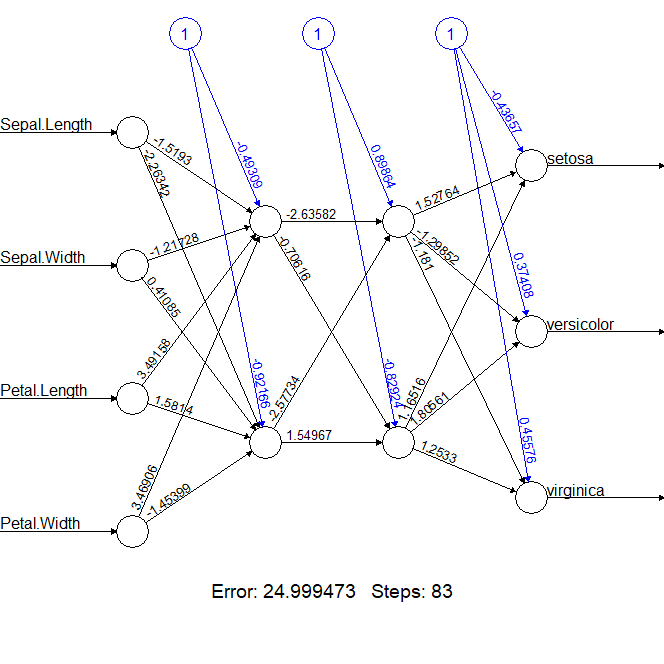``````
# 2个隐藏层，每层3个节点
> n3c <- neuralnet(Species~ Sepal.Length + Sepal.Width + Petal.Length + Petal.Width, + data = iris,hidden=c(3,3)) > n3c\$result.matrix
[,1]
error                     9.861760e-01
reached.threshold         9.760993e-03
steps                     1.842800e+04
Intercept.to.1layhid1     7.178501e-01
Sepal.Length.to.1layhid1  2.725448e-01
Sepal.Width.to.1layhid1   1.376482e+00
Petal.Length.to.1layhid1 -1.262652e+00
Petal.Width.to.1layhid1  -1.997635e+00
Intercept.to.1layhid2    -2.753156e+00
Sepal.Length.to.1layhid2 -1.465864e+00
Sepal.Width.to.1layhid2   4.125512e+00
Petal.Length.to.1layhid2 -6.026538e-01
Petal.Width.to.1layhid2   2.743376e+00
Intercept.to.1layhid3     1.379399e+00
Sepal.Length.to.1layhid3 -2.256887e-01
Sepal.Width.to.1layhid3   1.027688e+00
Petal.Length.to.1layhid3  3.221391e-01
Petal.Width.to.1layhid3  -2.847147e+00
Intercept.to.2layhid1    -2.353385e+00
1layhid1.to.2layhid1     -1.315389e+03
1layhid2.to.2layhid1      3.555585e+01
1layhid3.to.2layhid1      6.903238e+01
Intercept.to.2layhid2    -6.702997e-01
1layhid1.to.2layhid2      1.352140e+00
1layhid2.to.2layhid2     -5.184099e-02
1layhid3.to.2layhid2     -2.994567e-01
Intercept.to.2layhid3    -3.106167e+00
1layhid1.to.2layhid3      4.958443e+00
1layhid2.to.2layhid3     -2.185125e-01
1layhid3.to.2layhid3     -1.677417e+00
Intercept.to.setosa       4.026726e-01
2layhid1.to.setosa       -1.575905e-02
2layhid2.to.setosa       -1.461912e+00
2layhid3.to.setosa        2.965282e+00
Intercept.to.versicolor   6.790011e-01
2layhid1.to.versicolor   -9.706799e-01
2layhid2.to.versicolor    1.210438e+00
2layhid3.to.versicolor   -2.833834e+00
Intercept.to.virginica   -2.389590e-01
2layhid1.to.virginica     9.950653e-01
2layhid2.to.virginica     7.442383e-01
2layhid3.to.virginica    -3.957608e-01

# 画图
> plot(n3c)
``````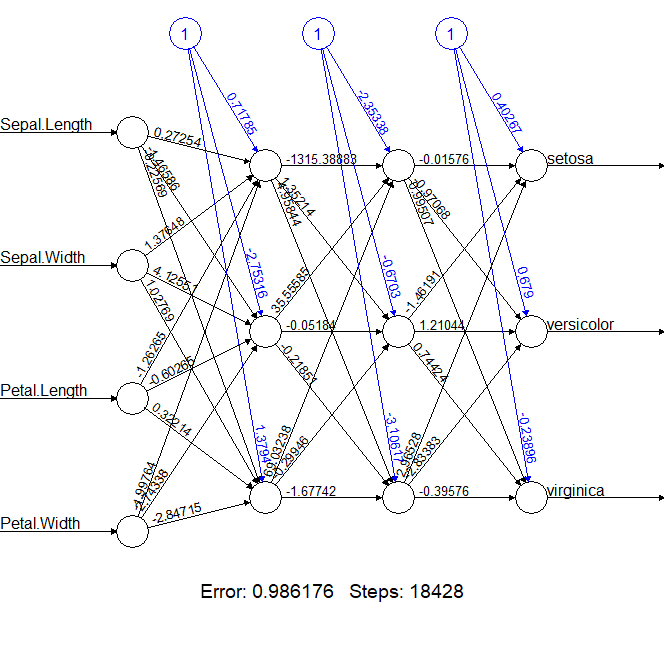## 7. 多层神经网络（深度学习）：多个隐藏层

``````
# 3个隐藏层，每层2个节点
> n3d <- neuralnet(Species~ Sepal.Length + Sepal.Width + Petal.Length +
Petal.Width, + data = iris,hidden=c(2,2,2))

# 结果矩阵
> n3d\$result.matrix
[,1]
error                     9.814611e-01
reached.threshold         9.856631e-03
steps                     2.599500e+04
Intercept.to.1layhid1     4.377454e+00
Sepal.Length.to.1layhid1  2.988690e+00
Sepal.Width.to.1layhid1   4.895009e+00
Petal.Length.to.1layhid1  4.739920e+00
Petal.Width.to.1layhid1   5.049575e+00
Intercept.to.1layhid2     1.713367e+00
Sepal.Length.to.1layhid2  4.900288e-01
Sepal.Width.to.1layhid2   6.486628e-01
Petal.Length.to.1layhid2 -1.317743e+00
Petal.Width.to.1layhid2  -1.286672e+00
Intercept.to.2layhid1    -5.678148e+01
1layhid1.to.2layhid1     -5.584444e+01
1layhid2.to.2layhid1      9.250502e+02
Intercept.to.2layhid2     1.417653e+01
1layhid1.to.2layhid2      1.370346e+01
1layhid2.to.2layhid2     -3.770254e+01
Intercept.to.3layhid1    -1.702465e+00
2layhid1.to.3layhid1      1.833016e+00
2layhid2.to.3layhid1      4.370202e+00
Intercept.to.3layhid2    -2.525917e+00
2layhid1.to.3layhid2      8.186075e+00
2layhid2.to.3layhid2      1.849959e+00
Intercept.to.setosa       1.991064e+00
3layhid1.to.setosa       -2.193503e+00
3layhid2.to.setosa        1.780463e-01
Intercept.to.versicolor  -2.462871e+00
3layhid1.to.versicolor    2.184454e+00
3layhid2.to.versicolor    1.303726e+00
Intercept.to.virginica    1.471821e+00
3layhid1.to.virginica     9.088568e-03
3layhid2.to.virginica    -1.481810e+00

# 画图
> plot(n3d)
``````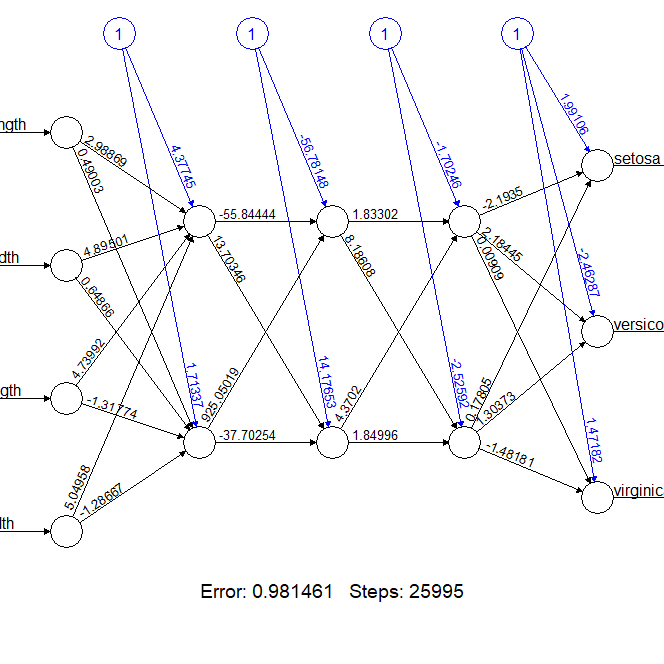## 知识图谱：最短路径算法Bellman-Ford

• 张丹, 分析师/程序员/Quant: R,Java,Nodejs
• blog: http://blog.fens.me
• email: bsspirit@gmail.com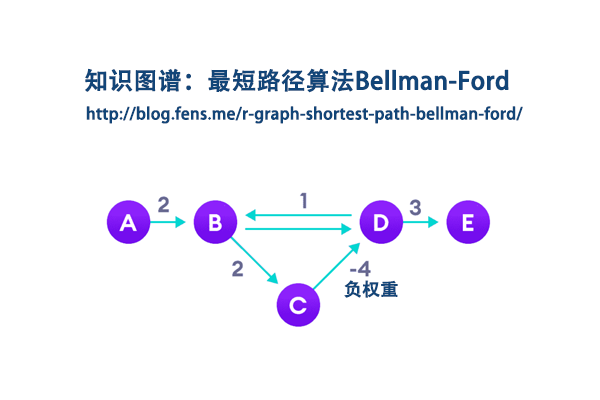1. Bellman-Ford算法介绍
2. R语言调用Bellman-Ford算法
3. 自己手写Bellman-Ford算法
4. 算一个复杂点的网络关系图

## 1. Bellman-Ford算法介绍

Bellman Ford算法最初于1955年被Alfonso Shimbel提出，但最终基于1958和1956年发表论文的 Richard Bellman和Lester Ford, Jr.二人命名。Edward F. Moore于1957年也提出了该算法，因此该算法有时也称作是Bellman Ford Moore算法。

Bellman-Ford算法与Dijkstra算法一样，解决的是单源最短路径问题。两者不同之处在于，Dijkstra只适用于无负权边的图，而Bellman-Ford算法无此限制：无论是否有向，无论是否有负权边，只要图中没有负权环，则该算法可以正确地给出起点到其余各点的最短路径，否则报告负权环的存在。

Bellman-Ford 算法采用动态规划进行设计，实现的时间复杂度为 O(V*E)，其中 V 为顶点数量，E 为边的数量。每次松弛操作实际上是对相邻节点的访问(相当于广度优先搜索)，第n次松弛操作保证了所有深度为n的路径最短。由于图的最短路径最长不会经过超过|V| – 1条边，所以可知贝尔曼-福特算法所得为最短路径，也可只时间复杂度为O(VE)。

Bellman-Ford 算法描述：

• 创建源顶点 v 到图中所有顶点的距离的集合 distSet，为图中的所有顶点指定一个距离值，初始均为 Infinite，源顶点距离为 0；
• 计算最短路径，执行 V – 1 次遍历；
• 对于图中的每条边：如果起点 u 的距离 d 加上边的权值 w 小于终点 v 的距离 d，则更新终点 v 的距离值 d；
• 检测图中是否有负权边形成了环，遍历图中的所有边，计算 u 至 v 的距离，如果对于 v 存在更小的距离，则说明存在环；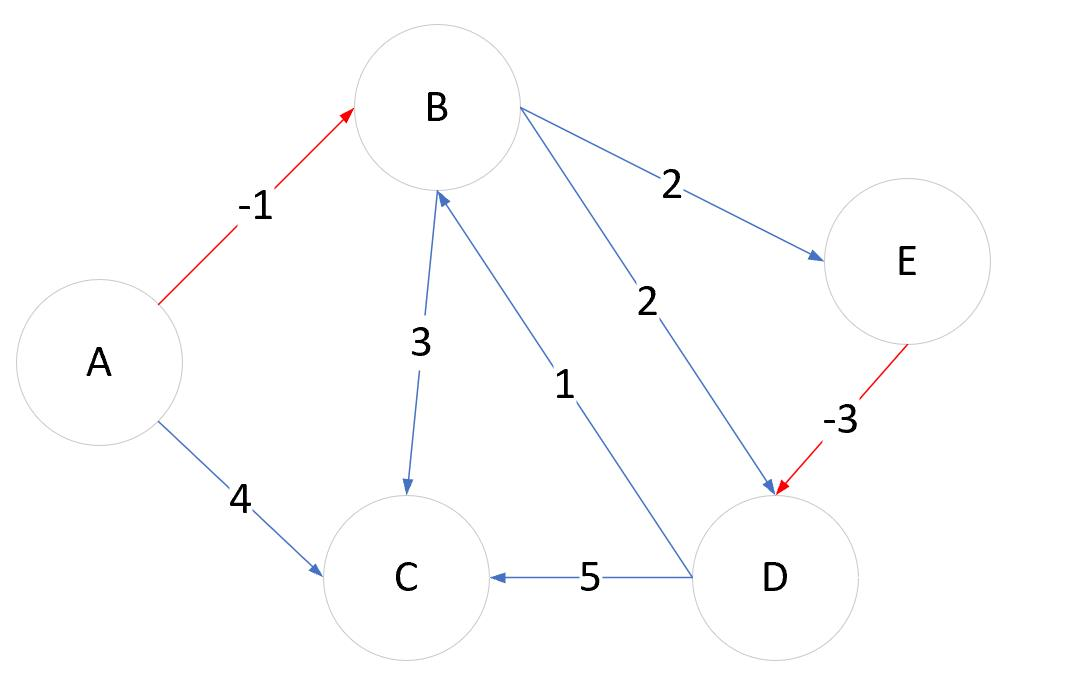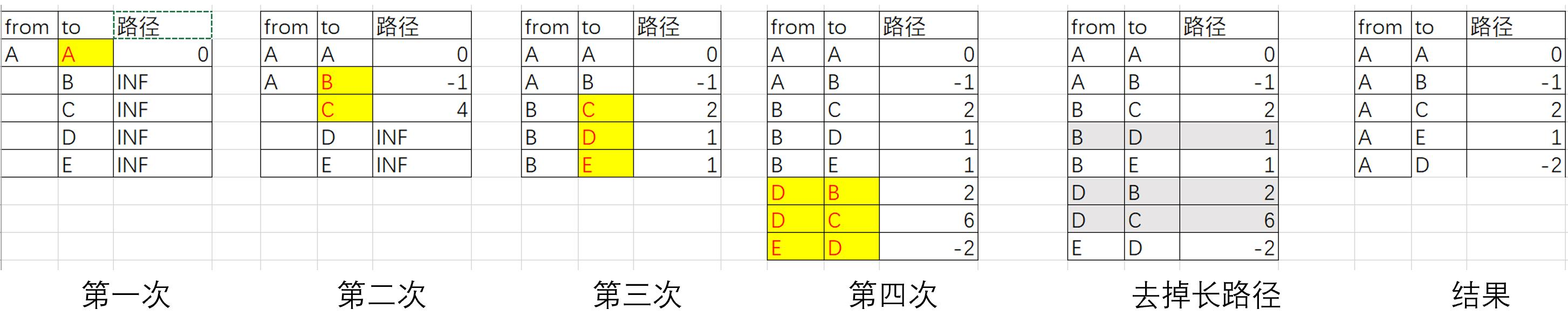## 2. R语言调用Bellman-Ford算法

``````# 加载igraph库
> library(igraph)

# 新建图数据
> df<-data.frame(
+   from=c("A","A","B","B","B","D","D","E"),
+   to=c("B","C","C","D","E","B","C","D"),
+   weight=c(-1,4,3,2,2,1,5,-3)
+ )

# 用igraph画图
> a<-graph_from_data_frame(df, directed = TRUE)
> E(a)\$arrow.size<-1
> E(a)\$arrow.width<-0.2
> E(a)\$label<-df\$weight

# layout需要设置一下，不然图的展示会很不好看
> plot(a,layout=layout.gem)
``````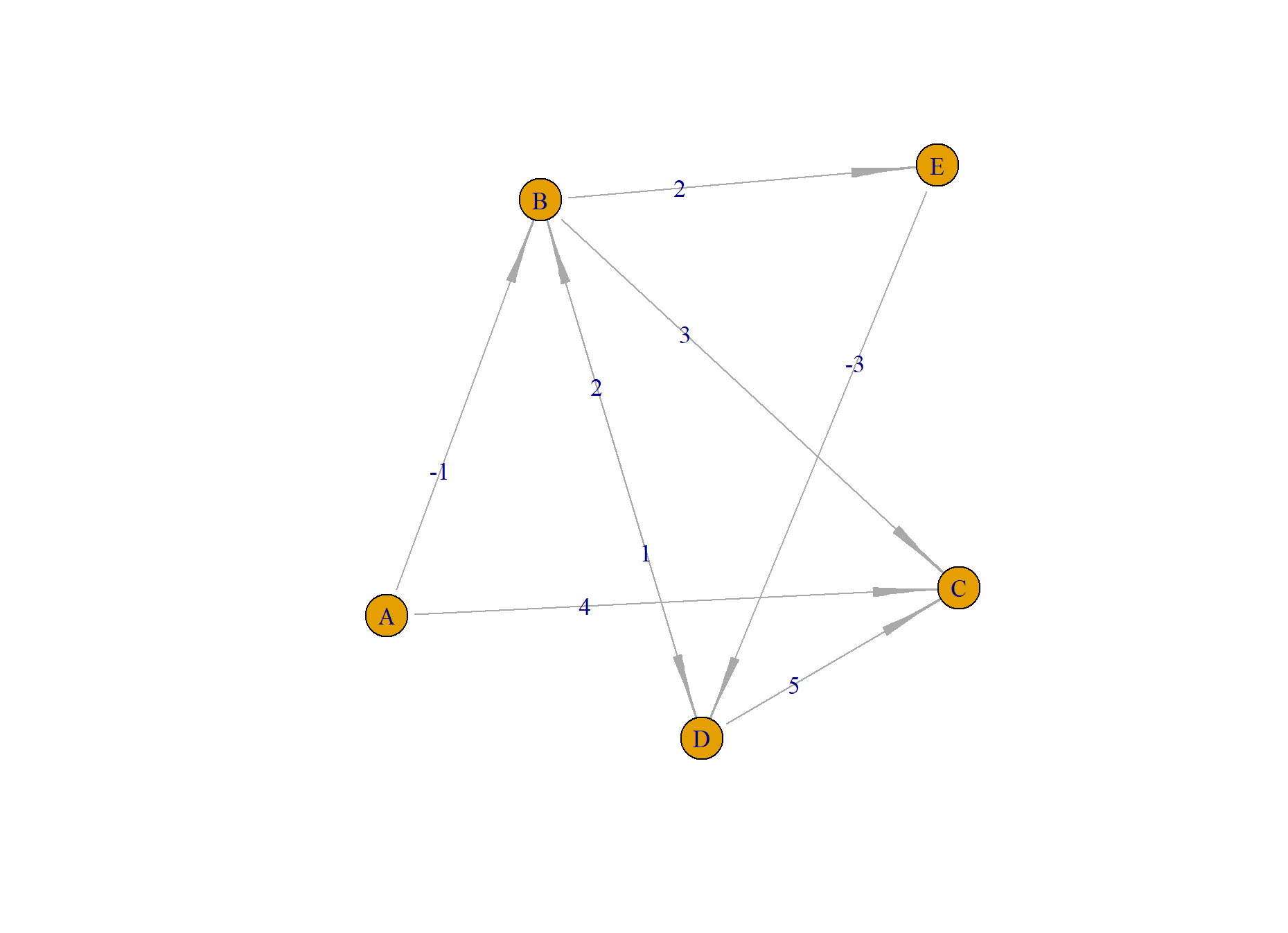layout需要设置一下，不然图的展示会节点叠加在一起，就看不出来图的样子了，我这里选择layout.gem类型。

``````# 使用bellman-ford算法
> shortest.paths(a,"A", algorithm = "bellman-ford")
Error in shortest.paths(a, "A", algorithm = "bellman-ford") :
At core/paths/bellman_ford.c:157 : cannot run Bellman-Ford algorithm, Negative loop detected while calculating shortest paths
``````

## 3. 自已手写Bellman-Ford算法

``````# bellman-ford 最短距离算法
# @param df数据集
# @param start开始节点
bellman_ford<-function(df,start="A"){

# 初始化结果数据集
rs<-data.frame(from=start,to=start,weight=0)

# 初始化结束节点
finishnode<-c()

# 初始化开始节点
nodes<-rs

# 当开始节点数量<结束节点数量时，继续循环计算
while(length(finishnode)<length(rs\$to)){

# 过滤已计算过的结束节点
if(length(finishnode)>0){
nodes<-rs[-which(rs\$to %in% finishnode),]
}

# 对原始数据进行循环，遍历每一行
for(i in 1:nrow(df)){
row<-df[i,];row

# 对结束节点进行循环，遍历每一个结束节点
for(j in nodes\$to){
if(row\$from==j){
# 把之前结束节点的权重叠加本次计算中
row\$weight<-row\$weight+rs\$weight[which(rs\$to==j)]
# 把结束节点加入到结果数据集
rs<-rbind(rs,row)
}
}
}

# 保留最短的唯一结束节点
rs2<-rs[order(rs\$weight),]
n<-which(duplicated(rs2\$to))
if(length(n)<0){
rs2<-rs2[-n,]
rs<-rs2[order(as.numeric(row.names(rs2))),]
}

# 更新已经完成的结束节点
finishnode<-c(finishnode,nodes\$to)
}
return(rs)
}
``````

``````> rs<-bellman_ford(df,"A")
> rs
from to weight
1    A  A      0
2    A  B     -1
3    B  C      2
5    B  E      1
8    E  D     -2
``````

``````# 计算2点间的最短路径
# @param rs 结果数据集
# @param start 开始节点
# @param end 结束节点
get_shortest_path<-function(rs,start,end){

# 初始化节点
q<-c(start)
idx<-which(rs\$from==start)

# 判断有几个分支
if(length(idx)>0){
for(i in idx){
# 递归计算
q2<-get_shortest_path(rs,rs\$to[i],end)
if(tail(q2,1)==end){
q<-c(q,q2)
}
}
}

# 查看队列状态
# print(q)

# 当找到结束节点，程序停止
if(tail(q,1)==end){
return(q)
}
return(q)
}

# 计算从开始节点到结束节点的路径
> path<-get_shortest_path(rs[-1,],"A","D")
> path
 "A" "B" "E" "D"
``````

``````# 把路径合并成data.frame
> path2<-data.frame(from=path[-length(path)],to=path[-1])
# 找到最短路径的索引
> idx<-which(paste0(df\$from,"-",df\$to) %in% paste0(path2\$from,"-",path2\$to))

# 涂色
> df\$color<-"gray"
> df\$color[idx]<-"red"
> E(a)\$color<-df\$color
> plot(a,layout=layout.gem)
``````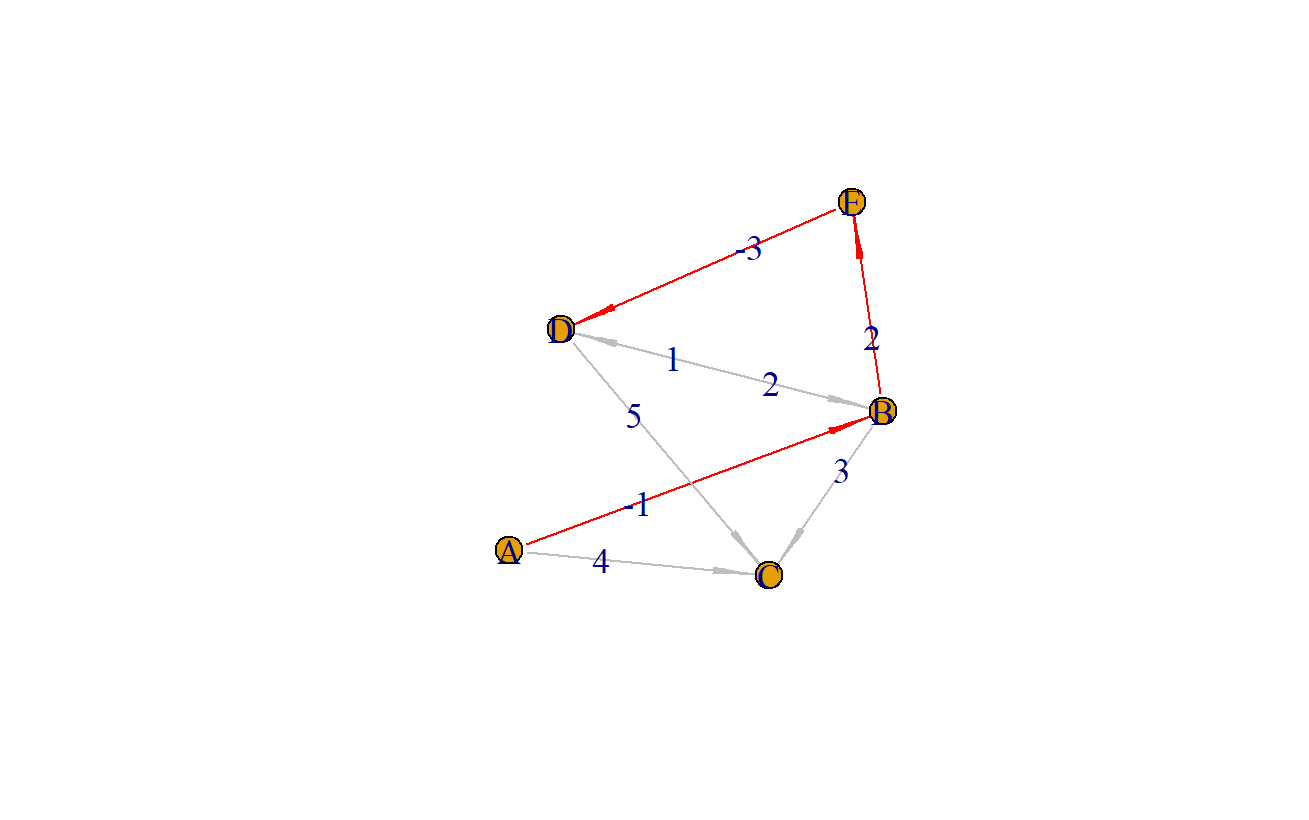## 4. 算一个复杂点的网路关系图

``````> df2<-data.frame(
+   from=c("A","B","C","D","C","F","B","B","F","G","H","F","E"),
+   to=  c("B","C","D","C","E","E","F","G","G","H","I","I","J"),
+   weight=c(5,20,10,-5,75,25,30,60,5,-50,-10,50,100)
+ )
``````

``````> a2<-graph_from_data_frame(df2, directed = TRUE)
> E(a2)\$arrow.size<-1
> E(a2)\$arrow.width<-0.2
> E(a2)\$label<-df2\$weight
> plot(a2,layout=layout.gem)
``````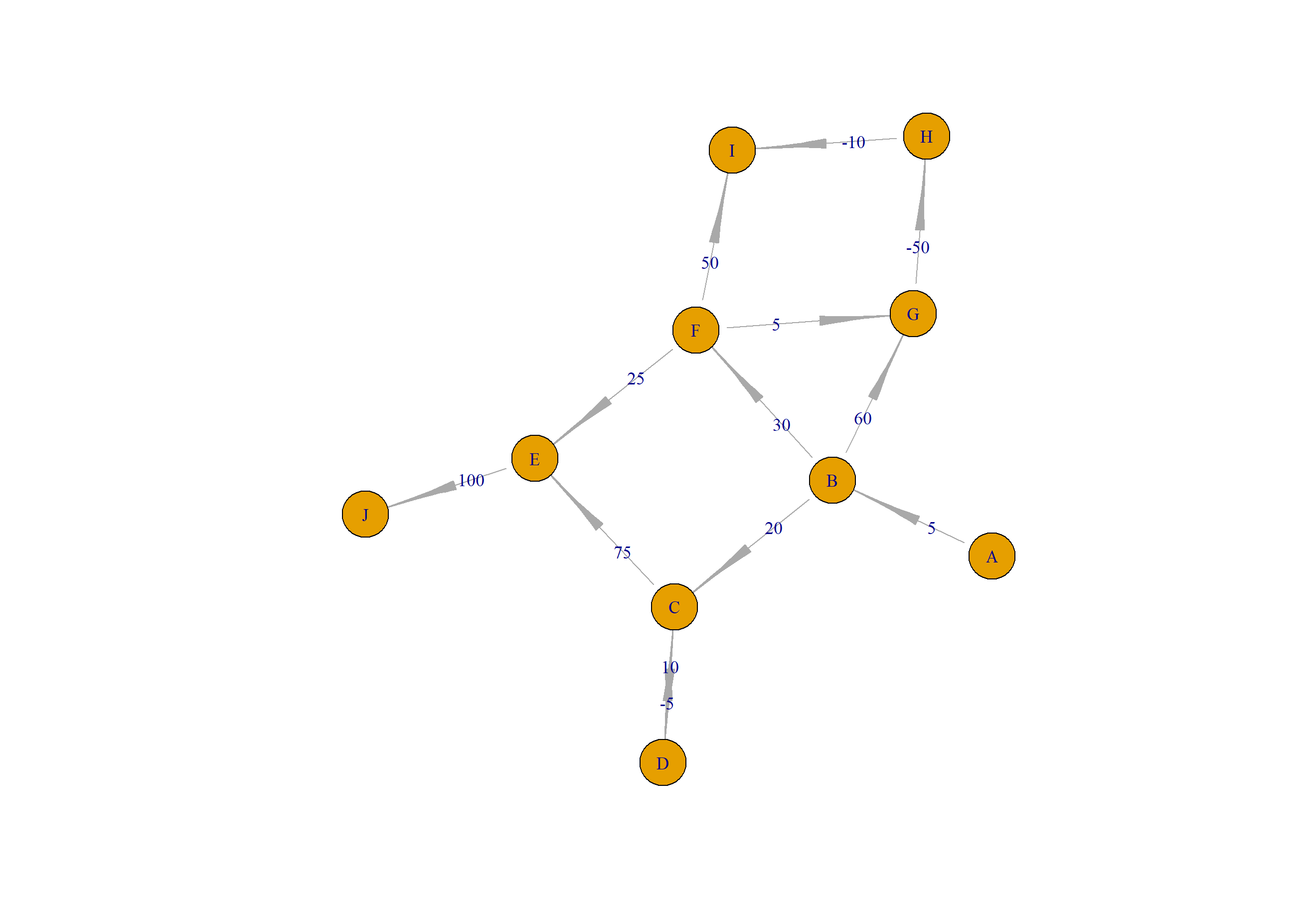``````> rs<-bellman_ford(df2,"A")
> rs
from to weight
1     A  A      0
2     A  B      5
3     C  D     35
6     F  E     60
7     B  F     35
9     F  G     40
10    G  H    -10
11    H  I    -20
13    E  J    160
21    B  C     25
``````

``````# 计算 A 到 D的最短路径
> path<-get_shortest_path(rs[-1,],"A","D")
> path
 "A" "B" "C" "D"

# 画图
> path2<-data.frame(from=path[-length(path)],to=path[-1])
> idx<-which(paste0(df2\$from,"-",df2\$to) %in% paste0(path2\$from,"-",path2\$to))
> df2\$color<-"gray"
> df2\$color[idx]<-"red"
> E(a2)\$arrow.size<-1
> E(a2)\$arrow.width<-0.2
> E(a2)\$color<-df2\$color
> plot(a2,layout=layout.gem)
``````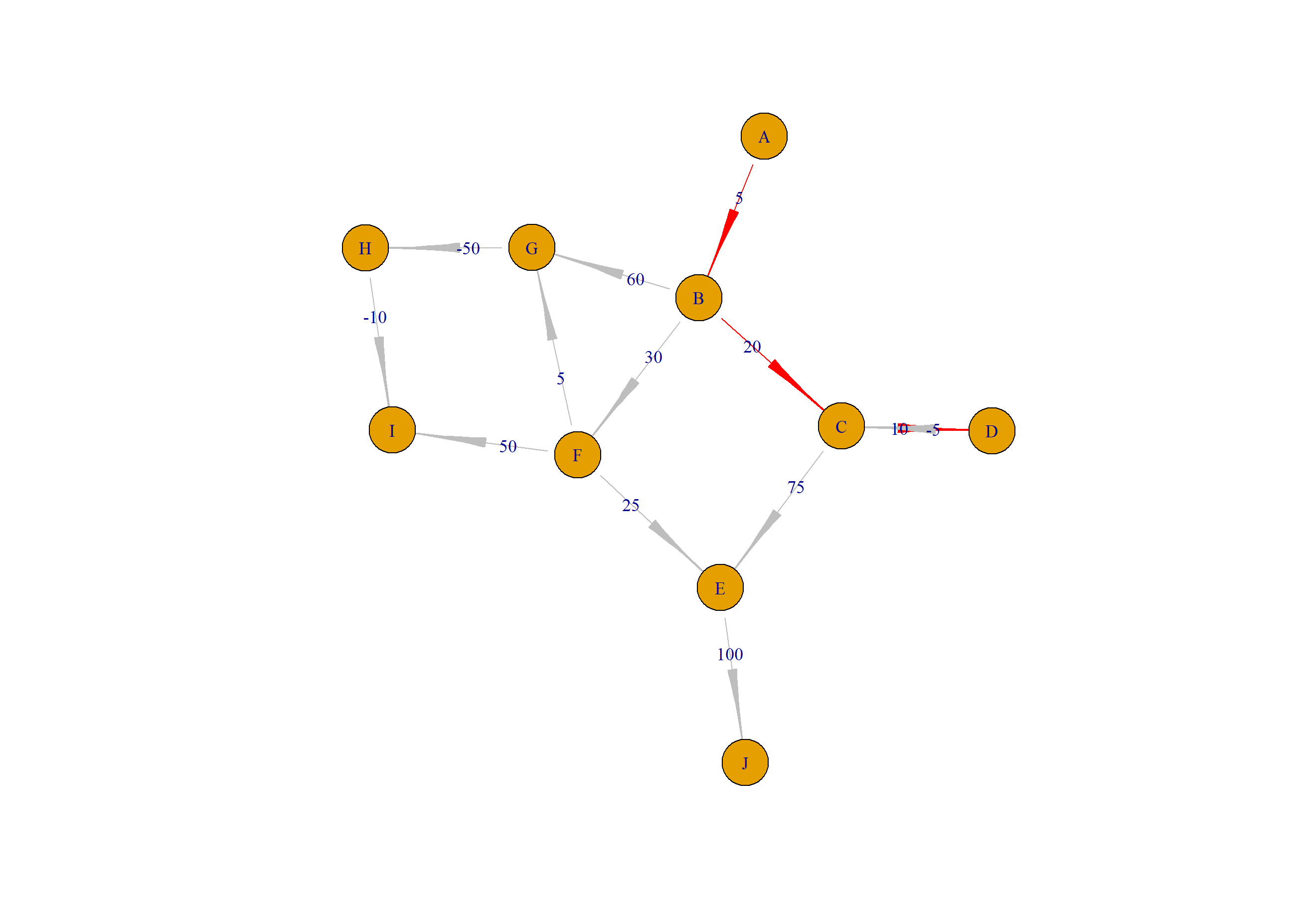``````# 计算 A 到 I的最短路径
> path<-get_shortest_path(rs[-1,],"A","I")
> path
 "A" "B" "F" "G" "H" "I"

# 画图
> path2<-data.frame(from=path[-length(path)],to=path[-1])
> idx<-which(paste0(df2\$from,"-",df2\$to) %in% paste0(path2\$from,"-",path2\$to))
> df2\$color<-"gray"
> df2\$color[idx]<-"red"
> E(a2)\$arrow.size<-1
> E(a2)\$arrow.width<-0.2
> E(a2)\$color<-df2\$color
> plot(a2,layout=layout.gem)
``````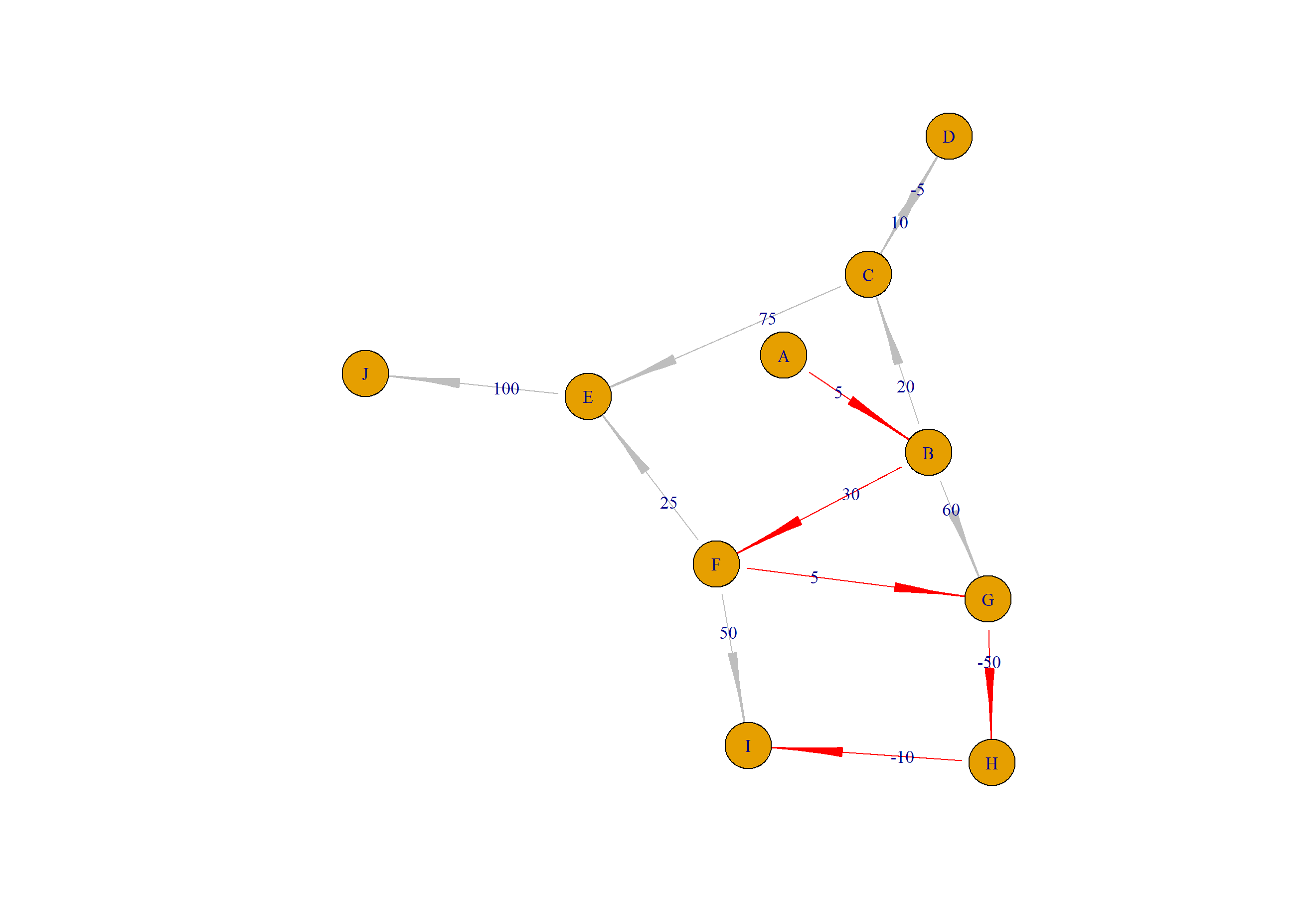http://blog.fens.me/r-graph-shortest-path-bellman-ford/## 知识图谱：最短路径算法Dijkstra

• 张丹, 分析师/程序员/Quant: R,Java,Nodejs
• blog: http://blog.fens.me
• email: bsspirit@gmail.com1. 最短路径算法介绍
2. Dijkstra 算法原理
3. 用R语言调用 Dijkstra 算法

## 1. 最短路径算法介绍

• 确定起点的最短路径问题，即给定起始节点，求该节点到其他剩余节点的最短路径，适合使用Dijkstra算法
• 确定终点的最短路径问题，即给定终点，求其他节点到该终点的最短路径。在无向图中，该问题与确定起点的问题等价；在有向图中，问题等价于把所有路径方向反转的确定起点的问题；
• 确定起点终点的最短路径问题，即给定起点和终点，求两节点之间的最短路径；
• 全局最短路径问题，即求图中所有节点之间的最短路径，适合使用Floyd-Warshall算法。

Dijkstra

Bellman-Ford

（Floyd-Warshall）

（Johnson）

## 2. Dijkstra 算法原理

Dijkstra算法，于1956年由荷兰计算机科学家艾兹赫尔.戴克斯特拉提出，用于解决赋权有向图的单源最短路径问题。所谓单源最短路径问题是指确定起点，寻找该节点到图中任意节点的最短路径，算法可用于寻找两个城市中的最短路径或是解决著名的旅行商问题。

1. 通过Dijkstra计算图中的最短路径时，需要指定一个起点A。
2. 简历两个数组S和U，S用于记录已求出最短路径的顶点(以及相应的最短路径长度)，U用于记录还未求出最短路径的顶点(以及该顶点到起点A的距离)。
3. 初始时，数组S中只有起点A；数组U中是除起点A之外的顶点，并且数组U中记录各顶点到起点A的距离。如果顶点与起点A不相邻，距离为无穷大INF。
4. 从数组U中找出路径最短的点X，并将其加入到数组S中；同时，从数组U中移除顶点X。接着，更新数组U中的各顶点到起点A的距离。
5. 重复第4步操作，直到遍历完所有顶点。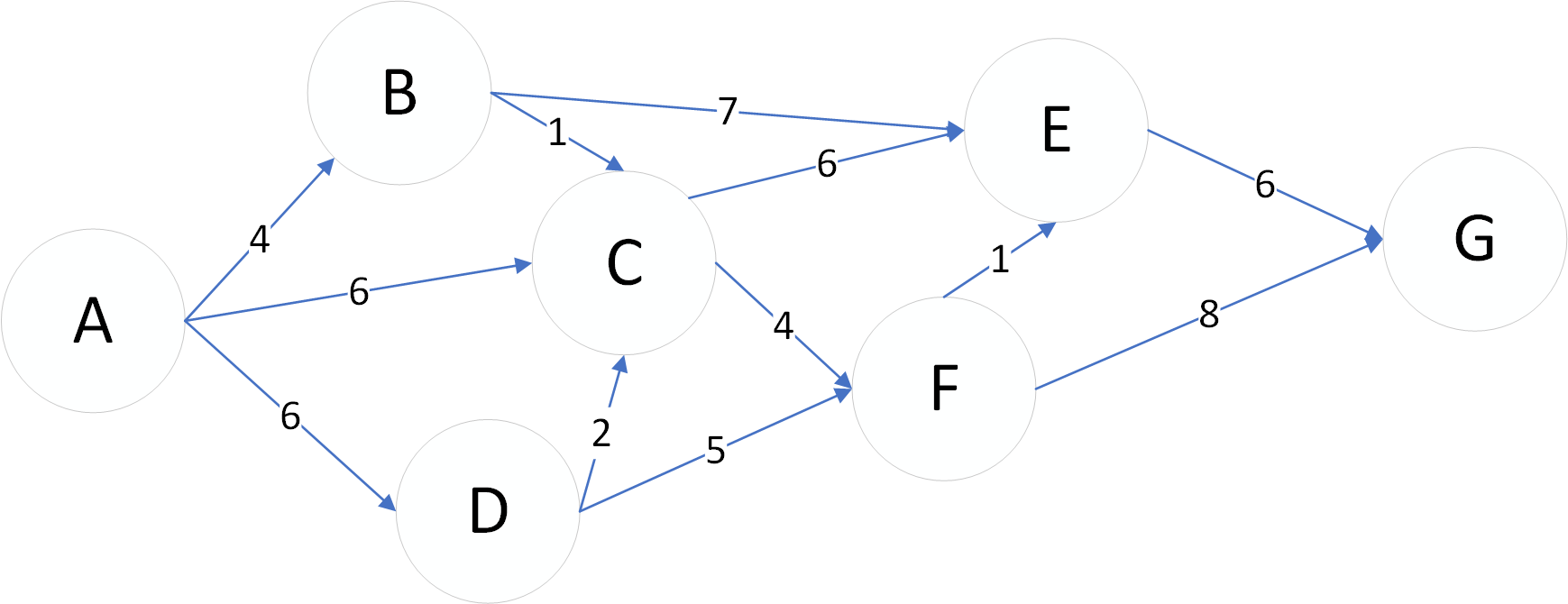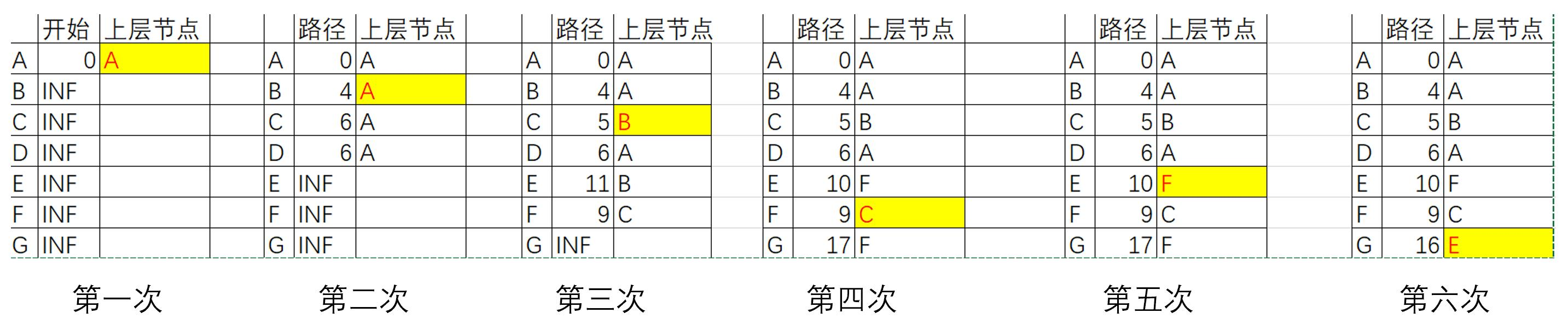## 3. 用R语言调用 Dijkstra 算法

``````> install.pacakges("igraph")
> library(igraph)
``````

``````> df<-data.frame(
+   from=c("A","A","A","B","D","B","C","D","C","F","E","F"),
+   to=c("B","D","C","C","C","E","E","F","F","E","G","G"),
+   weight=c(4,6,6,1,2,7,6,5,4,1,6,8)
+ )
> df
from to weight
1     A  B      4
2     A  D      6
3     A  C      6
4     B  C      1
5     D  C      2
6     B  E      7
7     C  E      6
8     D  F      5
9     C  F      4
10    F  E      1
11    E  G      6
12    F  G      8
``````

``````# 生成igraph对象
> a<-graph_from_data_frame(df, directed = TRUE, vertices = NULL)

# 画图
> E(a)\$arrow.size<-1
> E(a)\$arrow.width<-0.2
> E(a)\$label<-df\$weight
> plot(a)
``````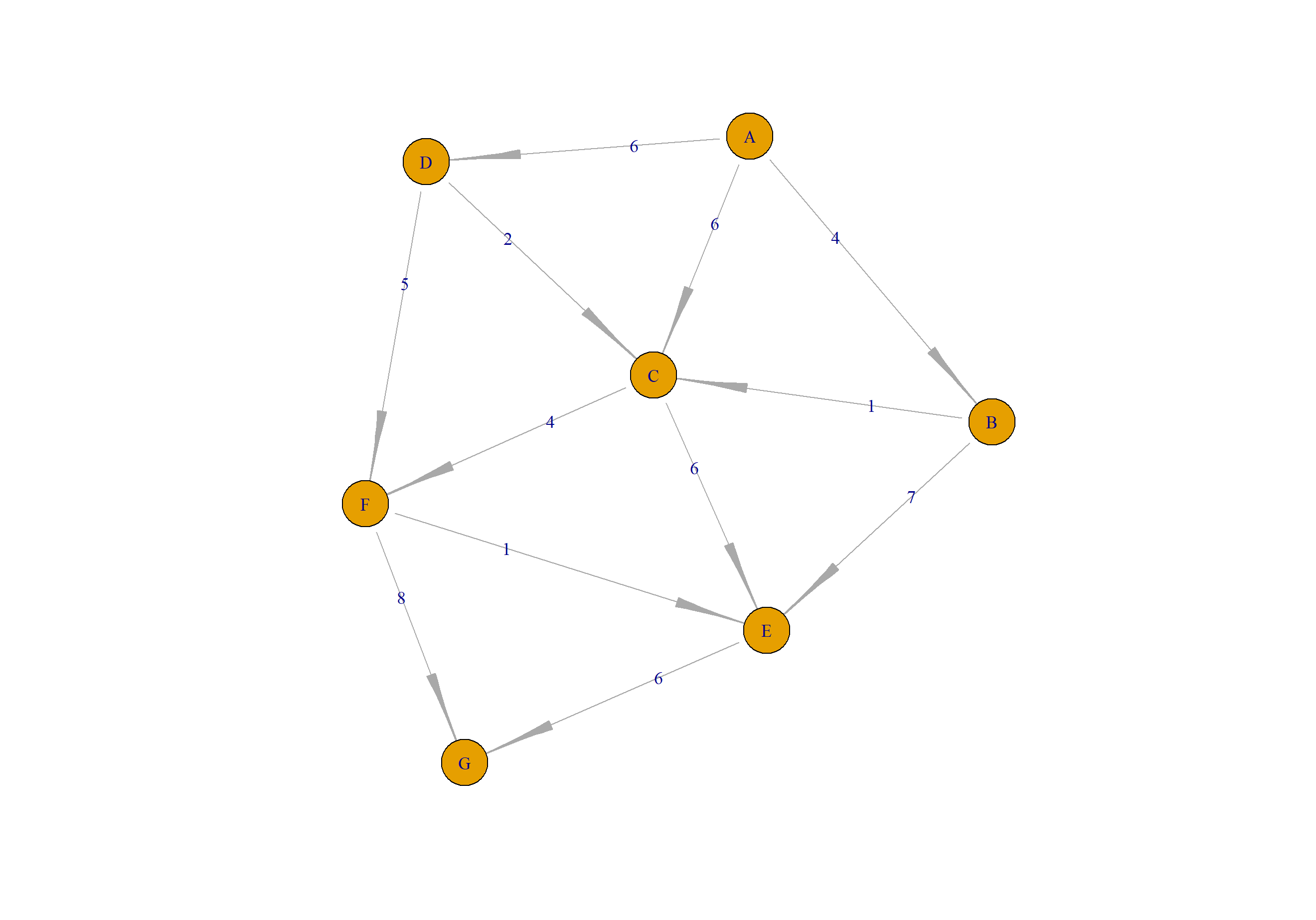``````# 通过最短路径的节点，建立边的关系
> s_vert<-data.frame(from=s_node[-length(s_node)],to=s_node[-1])

# 找到网络图中所有的边
> a_vert<-as_data_frame(a,what="edges")

# 找到最短路径的边的索引
> idx<-which(paste0(a_vert\$from,"-",a_vert\$to) %in% paste0(s_vert\$from,"-",s_vert\$to))
> idx
  1  4  9 10 11

# 涂色：红色为最短路径的边，灰色为非最短路径的边
> a_vert\$color<-"gray"
> a_vert\$color[idx]<-"red"

# 画图
> E(a)\$color<-a_vert\$color
> plot(a)
``````

``````> shortest.paths(a,"A", algorithm = "dijkstra")
A B D C F  E  G
A 0 4 6 5 9 10 16
``````

``````# 计算最短距离经过的点
> s_node<-all_shortest_paths(a,"A","G")\$res[]\$name
> snode
 "A" "B" "C" "F" "E" "G"

# 把节点进行涂色
> cols<-data.frame(label=V(a)\$name,color=0)
> cols\$color[which(cols\$label %in% s_node)]<-1
> cols
label color
1     A     1
2     B     1
3     D     0
4     C     1
5     F     1
6     E     1
7     G     1

# 画图
> V(a)\$color<-cols\$color
> plot(a)
``````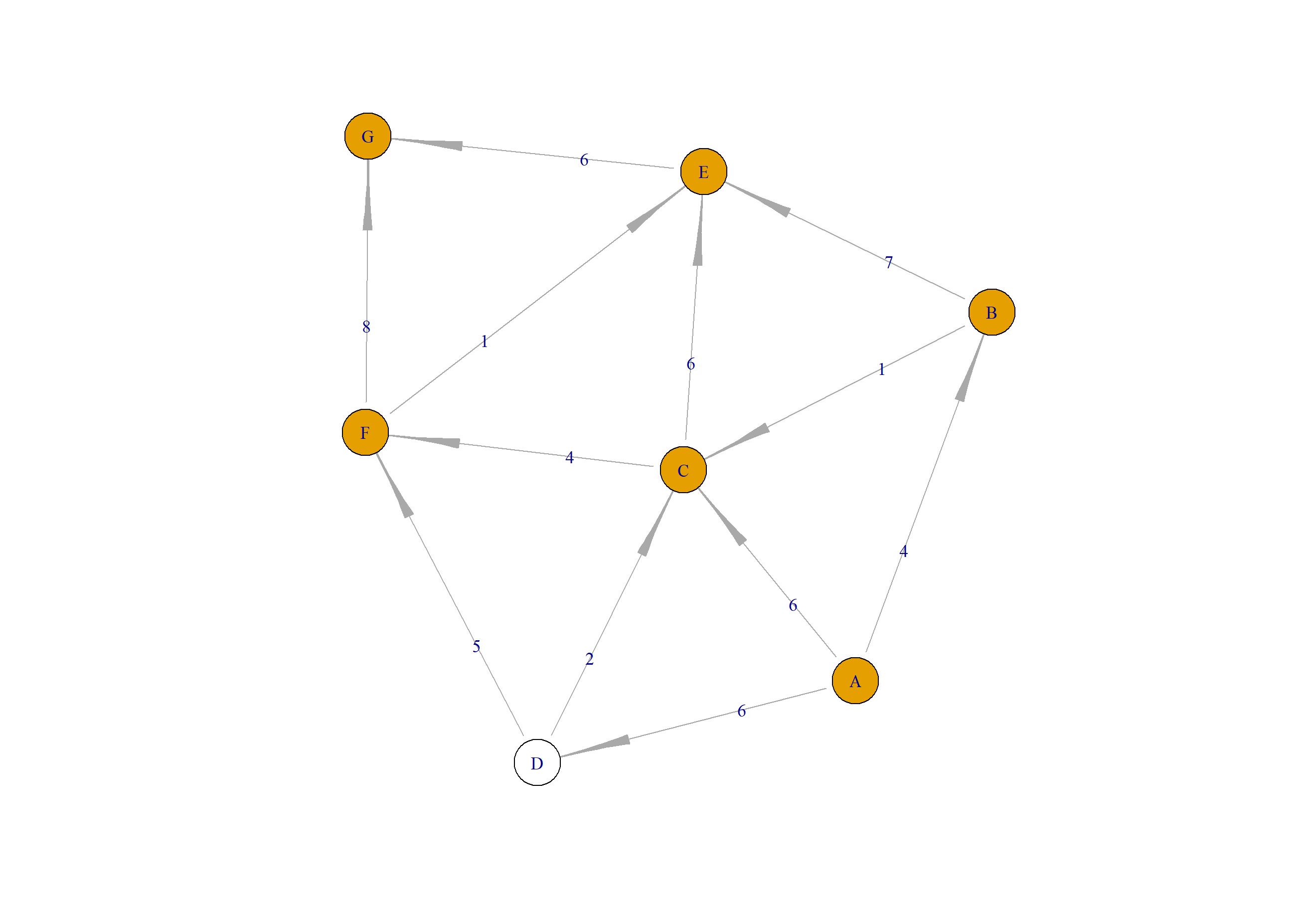``````# 建立最短路径节点的关系
> s_vert<-data.frame(from=s_node[-length(s_node)],to=s_node[-1])

# 找到网络图的完整路径
> a_vert<-as_data_frame(a,what="edges")

# 找到最短路径的边的索引
> idx<-which(paste0(a_vert\$from,"-",a_vert\$to) %in% paste0(s_vert\$from,"-",s_vert\$to))
> idx
  1  4  9 10 11

# 涂色
> a_vert\$color<-"gray"
> a_vert\$color[idx]<-"red"

# 画图
> E(a)\$color<-a_vert\$color
> plot(a)
``````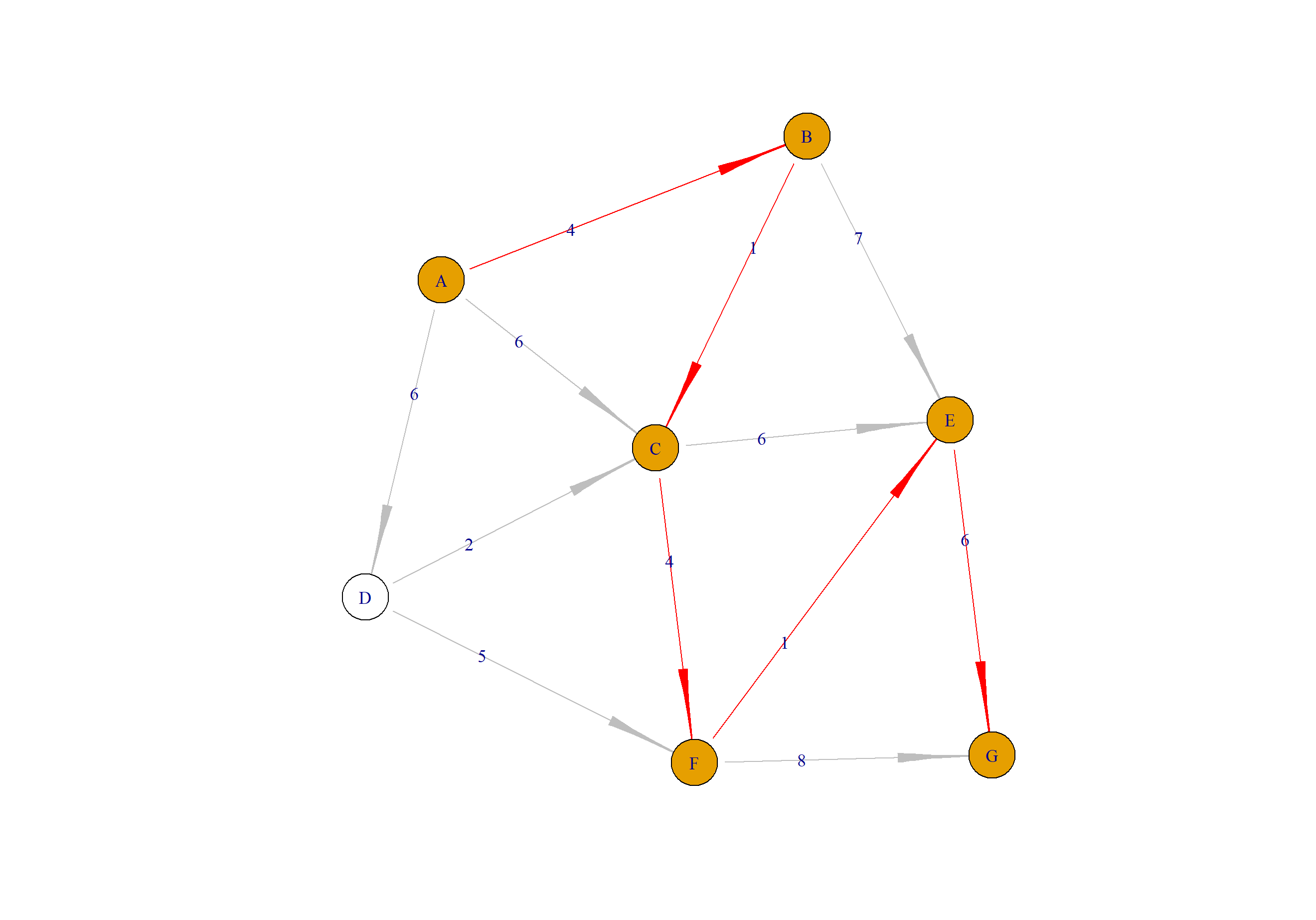``````> shortest.paths(a, algorithm = "dijkstra")
A  B  D  C F  E  G
A  0  4  6  5 9 10 16
B  4  0  3  1 5  6 12
D  6  3  0  2 5  6 12
C  5  1  2  0 4  5 11
F  9  5  5  4 0  1  7
E 10  6  6  5 1  0  6
G 16 12 12 11 7  6  0
``````

http://blog.fens.me/r-graph-shortest-path-dijkstra/## 线性和非线性的最小二乘回归

• 张丹(Conan), 程序员/Quant: Java,R,Nodejs
• blog: http://blog.fens.me
• email: bsspirit@gmail.com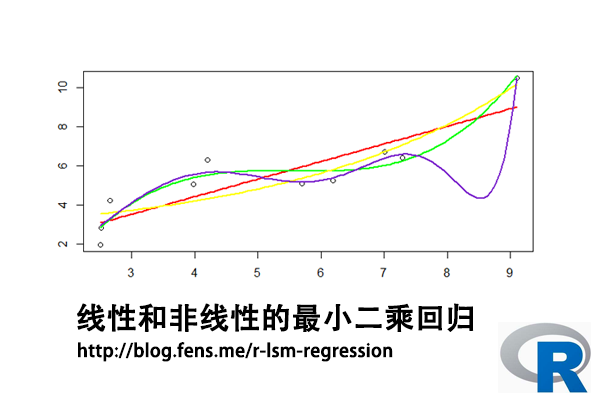1. 最小二乘法介绍
2. 最小二乘求解
3. 线性最小二乘回归
4. 非线性最小二乘回归

## 1. 最小二乘法介绍

• H, 目标值，观测值与理论值之差的平方和
• H’, 最小化的H值
• y, 观测值
• yi, 理论值
• argmin, 最小化函数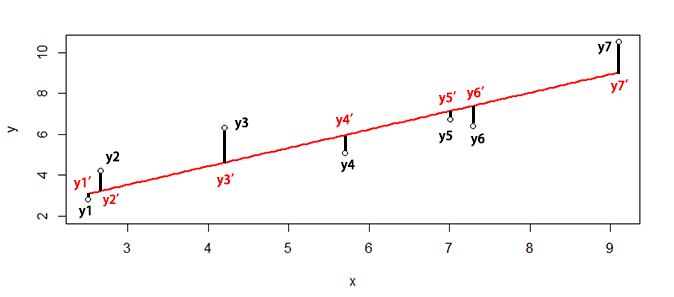``SSE=sum((y1-y1')^2+(y2-y2')^2+(y3-y3')^2+(y4-y4')^2+(y5-y5')^2+(y6-y6')^2+(y7-y7')^2)``

## 2. 最小二乘求解

``````> x<-c(6.19,2.51,7.29,7.01,5.7,2.66,3.98,2.5,9.1,4.2)
> y<-c(5.25,2.83,6.41,6.71,5.1,4.23,5.05,1.98,10.5,6.3)``````

``> plot(x,y)``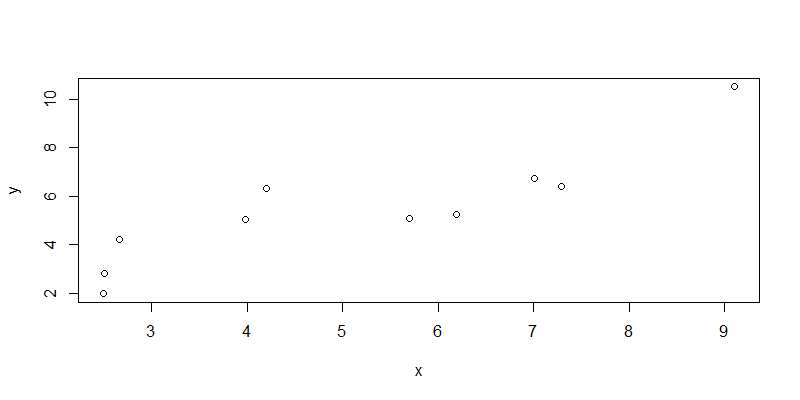``````
> fit<-lsfit(x,y);fit
\$coefficients
Intercept         X
0.8310557 0.9004584

\$residuals
 -1.1548933 -0.2612063 -0.9853975 -0.4332692 -0.8636686  1.0037250  0.6351198 -1.1022017
  1.4747728  1.6870190

\$intercept
 TRUE

\$qr
\$qt
 -17.1901414   6.2044421  -0.7047339  -0.1530724  -0.5856560   1.2766692   0.9102648
  -0.8295242   1.7584540   1.9625308

\$qr
Intercept           X
[1,] -3.1622777 -16.1718880
[2,]  0.3162278   6.8903149
[3,]  0.3162278  -0.2782874
[4,]  0.3162278  -0.2376506
[5,]  0.3162278  -0.0475287
[6,]  0.3162278   0.3936703
[7,]  0.3162278   0.2020970
[8,]  0.3162278   0.4168913
[9,]  0.3162278  -0.5409749
[10,]  0.3162278   0.1701682

\$qraux
 1.316228 1.415440

\$rank
 2

\$pivot
 1 2

\$tol
 1e-07

attr(,"class")
 "qr"
``````

• coefficients, 系数的最小二乘估计，包括截距Intercept和自变量X
• residuals, 残差
• intercept, 有截距
• qt, 矩阵QR分解。QR分解法是将矩阵分解成一个正规正交矩阵与上三角形矩阵。关于矩阵的详细操作，请参考文章R语言中的矩阵计算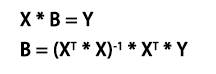• X，为自变量矩阵
• Y，为因变量矩阵
• B，为参数，需要求解
• XT，X的转置矩阵
• *, 矩阵乘法
• -1, 计算逆矩阵

``````
# 因变量矩阵
> y1<-matrix(y,ncol=1)

# 自变量矩阵，增加截距列
> x1<-matrix(c(x,rep(1,length(x))),ncol=2)

# 求解最小二乘解
> solve(t(x1)%*%x1) %*% t(x1) %*% y1
[,1]
[1,] 0.9004584
[2,] 0.8310557
``````

## 3. 线性最小二乘回归

``````
# 建立回归模型
> line<-lm(y~x)

# 查看回归模型
> summary(line)
Call:
lm(formula = y ~ x)

Residuals:
Min      1Q  Median      3Q     Max
-1.1549 -0.9550 -0.3472  0.9116  1.6870

Coefficients:
Estimate Std. Error t value Pr(>|t|)
(Intercept)   0.8311     0.9440   0.880 0.404338
x             0.9005     0.1698   5.302 0.000726 ***
---
Signif. codes:  0 ‘***’ 0.001 ‘**’ 0.01 ‘*’ 0.05 ‘.’ 0.1 ‘ ’ 1

Residual standard error: 1.17 on 8 degrees of freedom
Multiple R-squared:  0.7785,	Adjusted R-squared:  0.7508
F-statistic: 28.12 on 1 and 8 DF,  p-value: 0.0007263

# 残差平方和
> sum(line\$residuals^2)
 10.95334
``````

``````
# 设置x的取值区间
> new <- data.frame(x = seq(min(x),max(x),len = 100))

# 预测结果
> pred<-predict(line,newdata=new)
``````

``````
> plot(x,y)
> lines(new\$x,pred,col="red",lwd=2)
``````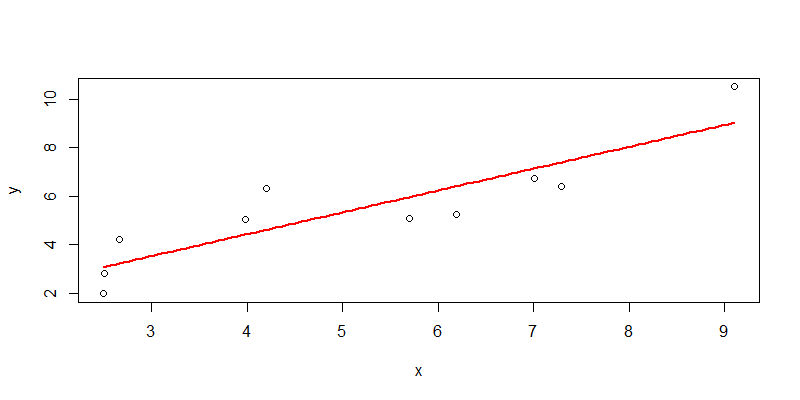## 4. 非线性最小二乘回归

4.1 一元二次函数非线性拟合

``````
# 建立回归模型
> nline1 <- nls(y ~ a*x+b*x^2+c, start = list(a = 1,b = 1,c=2))

# 查看模型结果
> nline1
Nonlinear regression model
model: y ~ a * x + b * x^2 + c
data: parent.frame()
a        b        c
0.008987 0.082188 2.850389
residual sum-of-squares: 9.758

Number of iterations to convergence: 1
Achieved convergence tolerance: 3.024e-06

# 分析模型
> summary(nline1)

Formula: y ~ a * x + b * x^2 + c

Parameters:
Estimate Std. Error t value Pr(>|t|)
a 0.008987   0.977890   0.009    0.993
b 0.082188   0.088760   0.926    0.385
c 2.850389   2.379763   1.198    0.270

Residual standard error: 1.181 on 7 degrees of freedom

Number of iterations to convergence: 1
Achieved convergence tolerance: 3.024e-06
``````

``````
# 模型预测
> npred1<-predict(nline1,newdata=new)

# 可视化
> plot(x,y)
> lines(new\$x,npred1,col="blue",lwd=2)
``````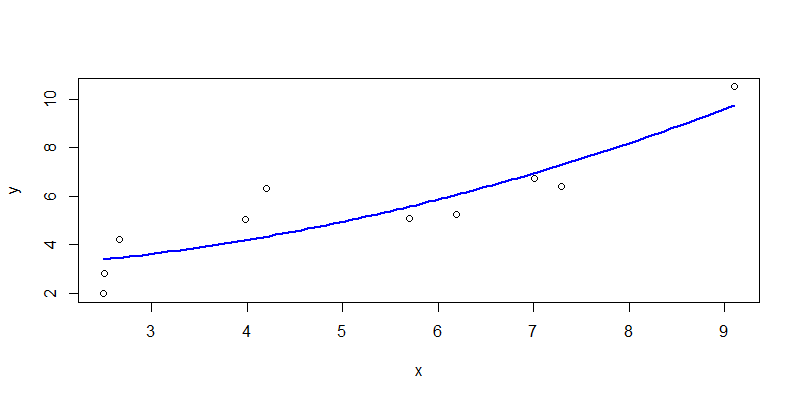4.2 一元三次函数非线性拟合

``````
> nline2 <- nls(y ~ a*x+b*x^2+c*x^3+d, start = list(a = 1,b = 1,c=2,d=1))

# 查看模型结果
> nline2
Nonlinear regression model
model: y ~ a * x + b * x^2 + c * x^3 + d
data: parent.frame()
a       b       c       d
10.011  -1.822   0.110 -12.516
residual sum-of-squares: 3.453

Number of iterations to convergence: 2
Achieved convergence tolerance: 3.983e-08

# 分析模型
> summary(nline2)

Formula: y ~ a * x + b * x^2 + c * x^3 + d

Parameters:
Estimate Std. Error t value Pr(>|t|)
a  10.01051    3.08630   3.244   0.0176 *
b  -1.82152    0.57797  -3.152   0.0198 *
c   0.10998    0.03323   3.310   0.0162 *
d -12.51586    4.88778  -2.561   0.0429 *
---
Signif. codes:  0 ‘***’ 0.001 ‘**’ 0.01 ‘*’ 0.05 ‘.’ 0.1 ‘ ’ 1

Residual standard error: 0.7586 on 6 degrees of freedom

Number of iterations to convergence: 2
Achieved convergence tolerance: 3.983e-08
``````

``````
> npred2<-predict(nline2,newdata=new)
> plot(x,y)
> lines(new\$x,npred2,col="green",lwd=2)
``````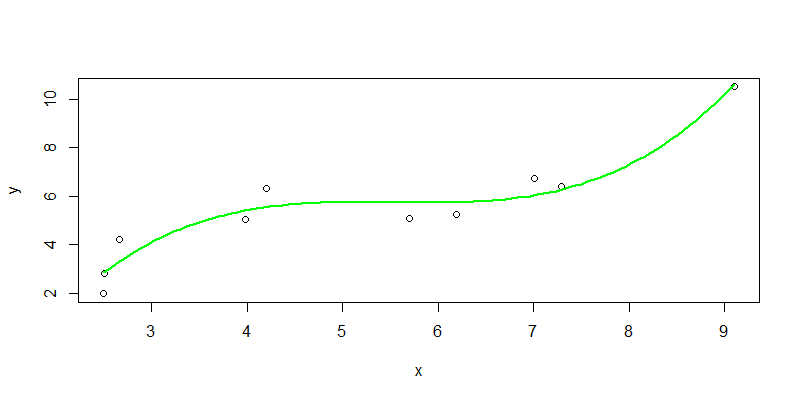4.3 指数函数非线性拟合

``````
> nline3 <- nls(y ~ a*exp(b*x)+c, start = list(a = 1,b = 1,c=2))

> nline3
Nonlinear regression model
model: y ~ a * exp(b * x) + c
data: parent.frame()
a      b      c
0.6720 0.2718 2.2087
residual sum-of-squares: 8.966

Number of iterations to convergence: 17
Achieved convergence tolerance: 7.136e-06

> summary(nline3)

Formula: y ~ a * exp(b * x) + c

Parameters:
Estimate Std. Error t value Pr(>|t|)
a   0.6720     1.2127   0.554    0.597
b   0.2718     0.1764   1.541    0.167
c   2.2087     2.2447   0.984    0.358

Residual standard error: 1.132 on 7 degrees of freedom

Number of iterations to convergence: 17
Achieved convergence tolerance: 7.136e-06
``````

``````
> npred3<-predict(nline3,newdata=new)
> plot(x,y)
> lines(new\$x,npred3,col="yellow",lwd=2)
``````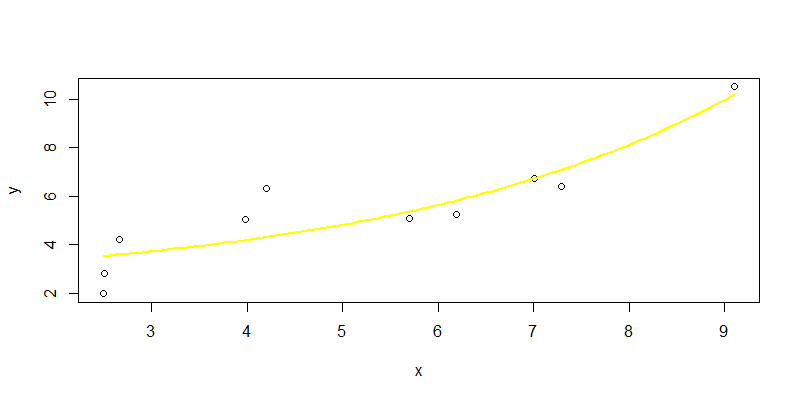4.4 倒数函数非线性拟合

``````> nline4 <- nls(y ~ b/x+c, start = list(b = 1,c=2))

# 查看模型结果
> nline4
Nonlinear regression model
model: y ~ b/x + c
data: parent.frame()
b     c
-17.0   9.5
residual sum-of-squares: 15.74

Number of iterations to convergence: 1
Achieved convergence tolerance: 1.259e-07

> summary(nline4)

Formula: y ~ b/x + c

Parameters:
Estimate Std. Error t value Pr(>|t|)
b  -17.003      4.108  -4.139  0.00326 **
c    9.500      1.077   8.817 2.15e-05 ***
---
Signif. codes:  0 ‘***’ 0.001 ‘**’ 0.01 ‘*’ 0.05 ‘.’ 0.1 ‘ ’ 1

Residual standard error: 1.403 on 8 degrees of freedom

Number of iterations to convergence: 1
Achieved convergence tolerance: 1.259e-07
``````

``````
> npred4<-predict(nline4,newdata=new)
> plot(x,y)
> lines(new\$x,npred4,col="gray",lwd=2)
``````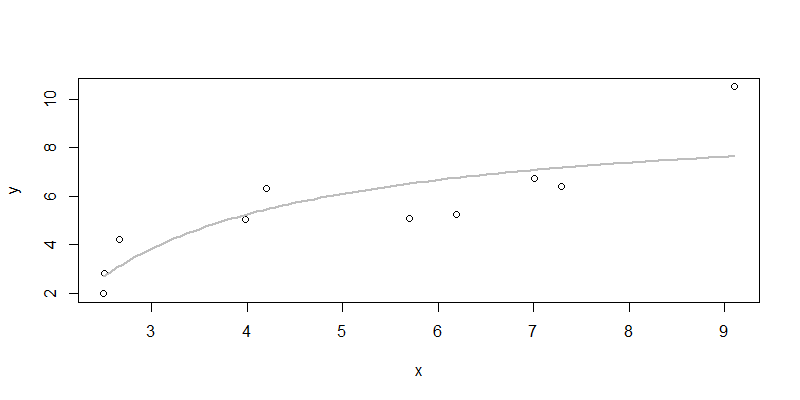4.5 模型优化

``````
> nline5 <- nls(y ~ a*x^3+b*x^5+c*x^7+d*x^9+e*x^11,
+               start = list(a=1,b=2,c=1,d=1,e=1))

# 查看模型结果
> nline5
Nonlinear regression model
model: y ~ a * x^3 + b * x^5 + c * x^7 + d * x^9 + e * x^11
data: parent.frame()
a          b          c          d          e
2.959e-01 -2.064e-02  5.837e-04 -7.324e-06  3.367e-08
residual sum-of-squares: 2.583

Number of iterations to convergence: 3
Achieved convergence tolerance: 2.343e-07

# 模型分析
> summary(nline5)

Formula: y ~ a * x^3 + b * x^5 + c * x^7 + d * x^9 + e * x^11

Parameters:
Estimate Std. Error t value Pr(>|t|)
a  2.959e-01  5.132e-02   5.767   0.0022 **
b -2.064e-02  6.176e-03  -3.341   0.0205 *
c  5.837e-04  2.468e-04   2.365   0.0644 .
d -7.324e-06  3.940e-06  -1.859   0.1222
e  3.367e-08  2.139e-08   1.574   0.1763
---
Signif. codes:  0 ‘***’ 0.001 ‘**’ 0.01 ‘*’ 0.05 ‘.’ 0.1 ‘ ’ 1

Residual standard error: 0.7187 on 5 degrees of freedom

Number of iterations to convergence: 3
Achieved convergence tolerance: 2.343e-07
``````

``````
> npred5<-predict(nline5,newdata=new)
> plot(x,y)
> lines(new\$x,npred5,col="purple3",lwd=2)
``````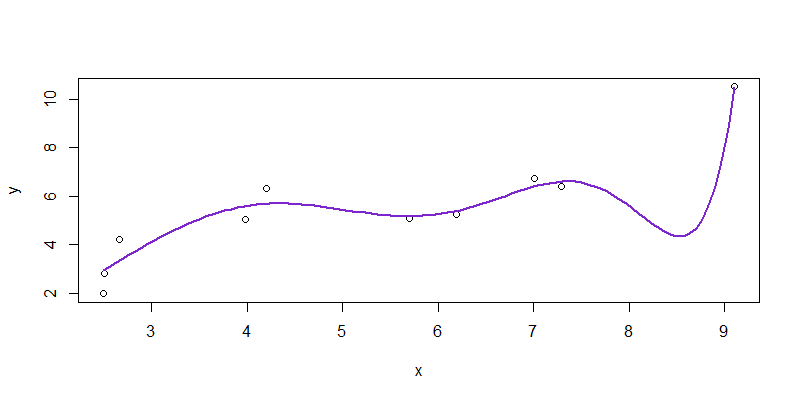4.6 总结

 拟合函数 残差平方和 参数T检验显著性 解释性 颜色 一次函数 10.95334 不显著 好 红色 二次函数 9.758 不显著 好 蓝色 三次函数 3.453 显著 不好 绿色 指数函数 8.966 不显著 不好 黄色 倒数函数 15.74 显著 好 灰色 高次函数 2.583 显著 无法解释 紫色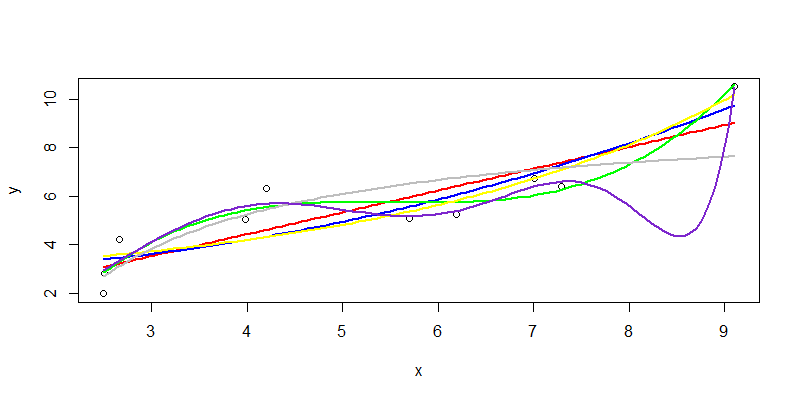## R语言实现聚类kmeans

R的极客理想系列文章，涵盖了R的思想，使用，工具，创新等的一系列要点，以我个人的学习和体验去诠释R的强大。

R语言作为统计学一门语言，一直在小众领域闪耀着光芒。直到大数据的爆发，R语言变成了一门炙手可热的数据分析的利器。随着越来越多的工程背景的人的加入，R语言的社区在迅速扩大成长。现在已不仅仅是统计领域，教育，银行，电商，互联网….都在使用R语言。

• 张丹(Conan), 程序员/Quant: Java,R,Nodejs
• blog: http://blog.fens.me
• email: bsspirit@gmail.com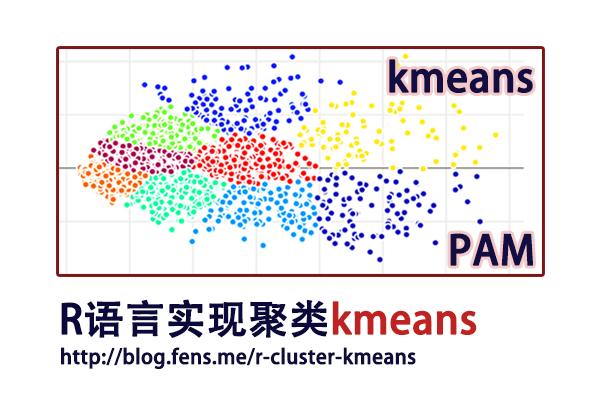1. k-means实现
2. PAM实现
3. 可视化和段剖面图

## 1. k-means实现

k-means算法，是一种最广泛使用的聚类算法。k-means以k作为参数，把数据分为k个组，通过迭代计算过程，将各个分组内的所有数据样本的均值作为该类的中心点，使得组内数据具有较高的相似度，而组间的相似度最低。

k-means工作原理：

1. 初始化数据，选择k个对象作为中心点。
2. 遍历整个数据集，计算每个点与每个中心点的距离，将它分配给距离中心最近的组。
3. 重新计算每个组的平均值，作为新的聚类中心。
4. 上面2-3步，过程不断重复，直到函数收敛，不再新的分组情况出现。

k-means聚类，适用于连续型数据集。在计算数据样本之间的距离时，通常使用欧式距离作为相似性度量。k-means支持多种距离计算，还包括maximum, manhattan, pearson, correlation, spearman, kendall等。各种的距离算法的介绍，请参考文章R语言实现46种距离算法

1.1 kmeans()函数实现

• Win10 64bit
• R: 3.4.4 x86_64-w64-mingw32

``````# 创建数据集
> set.seed(0)
> df <- rbind(matrix(rnorm(100, 0.5, 4.5), ncol = 2),
+             matrix(rnorm(100, 0.5, 0.1), ncol = 2))
> colnames(df) <- c("x", "y")
x          y
[1,]  6.1832943  1.6976181
[2,] -0.9680501 -1.1951622
[3,]  6.4840967 11.4861408
[4,]  6.2259319 -3.0790260
[5,]  2.3658865  0.2530514
[6,] -6.4297752  1.6256360
``````

``````
> cl <- kmeans(df,2); cl
K-means clustering with 2 clusters of sizes 14, 86

Cluster means:                   # 中心点坐标
x         y
1  5.821526 2.7343127
2 -0.315946 0.1992429

Clustering vector:               # 分组的索引
 1 2 1 1 2 2 2 2 2 1 1 2 2 2 2 2 2 2 2 2 2 2 2 1 2 1 1 2 2 2 2 2 2 2 2 1 1 2 1 2 1 2 2 2 2 2 2 1 1 2
 2 2 2 2 2 2 2 2 2 2 2 2 2 2 2 2 2 2 2 2 2 2 2 2 2 2 2 2 2 2 2 2 2 2 2 2 2 2 2 2 2 2 2 2 2 2 2 2 2 2

Within cluster sum of squares by cluster:
 316.0216 716.4009                       # withinss，分组内平方和
(between_SS / total_SS =  34.0 %)          # 组间的平方和/总平方和，用于衡量点聚集程度

Available components:            # 对象属性
 "cluster"      "centers"      "totss"        "withinss"     "tot.withinss" "betweenss"
 "size"         "iter"         "ifault"

# 查看数据分组情况，第1组86个，第2组14个
> cl\$size
 86 14
``````

• cluster，每个点的分组
• centers，聚类的中心点坐标
• totss，总平方和
• withinss，每个分组内的平方和
• tot.withinss，分组总和，sum(withinss)
• betweenss，组间的平方和，totss – tot.withinss
• size，每个组中的数据点数量
• iter，迭代次数。
• ifault，可能有问题的指标

1.2 kcca()函数实现

``````
# 安装flexclust包
> # install.packages("flexclust")
> library(flexclust)

# 进行聚类
> clk<-kcca(df,k=2);clk
kcca object of family ‘kmeans’

call:
kcca(x = df, k = 2)

cluster sizes:  # 聚类的分组大小
1  2
84 16

# 聚类的中心
> clk@centers
x         y
[1,] -0.3976465 0.2015319
[2,]  5.4832702 2.4054118

# 查看聚类的概览信息
> summary(clk)
kcca object of family ‘kmeans’

call:
kcca(x = df, k = 2)

cluster info:         # 每个组的基本信息，包括分组数量，平均距离、最大距离、分割值
size  av_dist max_dist separation
1   84 2.102458 9.748136   3.368939
2   16 3.972920 9.576635   3.189891

convergence after 5 iterations                   # 5次迭代
sum of within cluster distances: 240.1732        # 聚类距离之和
``````

``````> plot(df, col = cl\$cluster, main="Kmeans Cluster")
> points(cl\$centers, col = 1:3, pch = 10, cex = 4) # 画出kmeans()函数效果
``````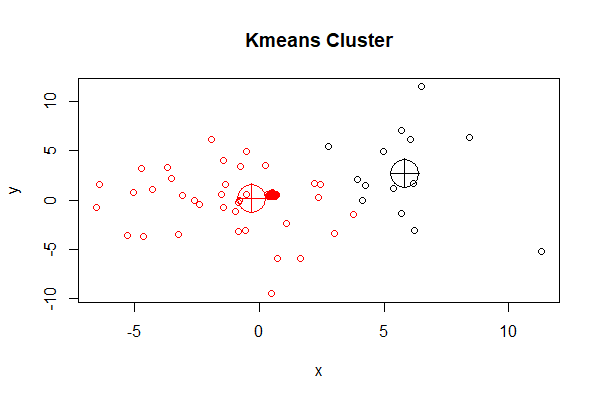``````> points(clk@centers, col = 3:4, pch = 10, cex = 4)  # 画出kcca()函数效果
``````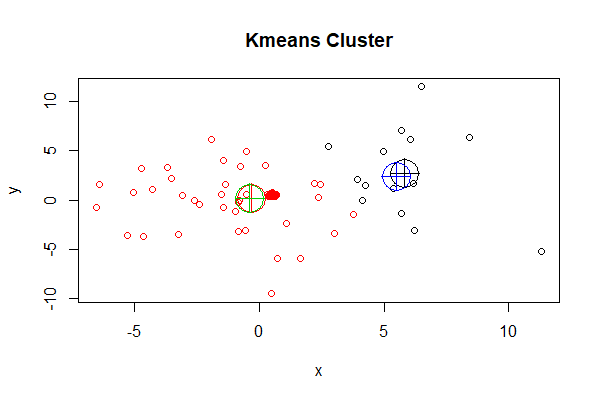k-means算法，也有一些缺点就是对于孤立点是敏感的，会被一些极端值影响聚类的效果。一种改进的算法是PAM，用于解决这个问题。PAM不使用分组平均值作为计算的参照点，而是直接使用每个组内最中心的对象作为中心点。

## 2. PAM实现

PAM(Partitioning Around Medoids)，又叫k-medoids，它可以将数据分组为k个组，k为数量是要事前定义的。PAM与k-means一样，找到距离中心点最小点组成同一类。PAM对噪声和异常值更具鲁棒性，该算法的目标是最小化对象与其最接近的所选对象的平均差异。PAM可以支持混合的数据类型，不仅限于连续变量。

PAM算法分为两个阶段：

1. 第1阶段BUILD，为初始集合S选择k个对象的集合。
2. 第2阶段SWAP，尝试用未选择的对象，交换选定的中心点，来提高聚类的质量。

PAM的工作原理：

1. 初始化数据集，选择k个对象作为中心。
2. 遍历数据点，把每个数据点关联到最近中心点m。
3. 随机选择一个非中心对象，与中心对象交换，计算交换后的距离成本
4. 如果总成本增加，则撤销交换的动作。
5. 上面2-4步，过程不断重复，直到函数收敛，中心不再改变为止。

• 消除了k-means算法对于孤立点的敏感性。
• 比k-means的计算的复杂度要高。
• 与k-means一样，必须设置k的值。
• 对小的数据集非常有效，对大数据集效率不高。

``````
> install.packages("cluster")
> library(cluster)
``````

pam()函数定义：

``````
pam(x, k, diss = inherits(x, "dist"), metric = "euclidean",
medoids = NULL, stand = FALSE, cluster.only = FALSE,
do.swap = TRUE,
keep.diss = !diss && !cluster.only && n < 100,
keep.data = !diss && !cluster.only,
pamonce = FALSE, trace.lev = 0)
``````

• x，数据框或矩阵，允许有空值(NA)
• k，设置分组数量
• diss，为TRUE时，x为距离矩阵；为FALSE时，x是变量矩阵。默认为FALSE
• metric，设置距离算法，默认为euclidean，距离矩阵忽略此项
• medoids，指定初始的中心，默认为不指定。
• stand，为TRUE时进行标准化，距离矩阵忽略此项。
• cluster.only，为TRUE时，仅计算聚类结果，默认为FALSE
• do.swap，是否进行中心点交换，默认为TRUE；对于超大的数据集，可以不进行交换。
• keep.diss，是否保存距离矩阵数据
• keep.data，是否保存原始数据
• pamonce，一种加速算法，接受值为TRUE,FALSE,0,1,2
• trace.lev，日志打印，默认为0，不打印

``````> kclus <- pam(df,2)

# 查看kclus对象
> kclus
Medoids:                                     # 中心点
ID         x         y
[1,] 27 5.3859621 1.1469717
[2,] 89 0.4130217 0.4798659

Clustering vector:                           # 分组
 1 2 1 1 2 2 2 2 2 1 1 2 2 2 2 2 2 2 2 2 2 2 2 1 2 1 1 2 2 2 2 2 2 2 1 1 1 2 1 2 1 2 2 2 2 2 2 1 1 2
 2 2 2 2 2 2 2 2 2 2 2 2 2 2 2 2 2 2 2 2 2 2 2 2 2 2 2 2 2 2 2 2 2 2 2 2 2 2 2 2 2 2 2 2 2 2 2 2 2 2

Objective function:                          # 目标函数的局部最小值
build     swap
2.126918 2.124185

Available components:                        # 聚类对象的属性
 "medoids"    "id.med"     "clustering" "objective"  "isolation"  "clusinfo"   "silinfo"
 "diss"       "call"       "data"

> kclus\$clusinfo        # 聚类的分组数量，每个组的平均距离、最大距离、分割值
size  max_diss  av_diss diameter separation
[1,]   15 10.397323 4.033095 17.35984   1.556862
[2,]   85  9.987604 1.787318 15.83646   1.556862
``````

• medoids，中心点的数据值
• id.med，中心点的索引
• clustering，每个点的分组
• objective，目标函数的局部最小值
• isolation，孤立的聚类(用L或L*表示)
• clusinfo，每个组的基本信息
• silinfo，存储各观测所属的类、其邻居类以及轮宽（silhouette）值
• diss，不相似度
• call，执行函数和参数
• data，原始数据集

``````# 画图
> plot(df, col = kclus\$clustering, main="Kmedoids Cluster")
> points(kclus\$medoids, col = 1:3, pch = 10, cex = 4)
``````

##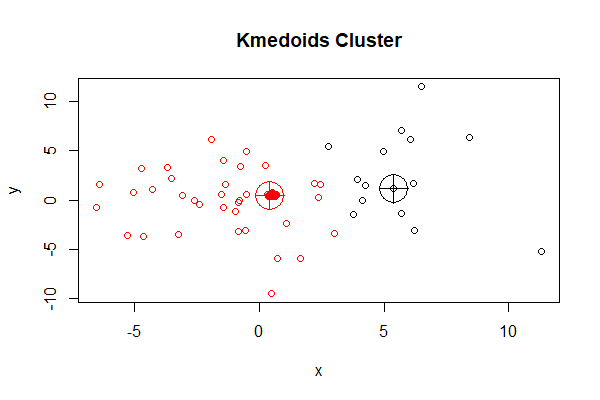``````
# 设置聚类的中心为1，2
> kclus2<-pam(df,2,medoids=c(1,2),trace.lev=20)
C pam(): computing 4951 dissimilarities from  100 x 2  matrix: [Ok]
pam()'s bswap(*, s=21.837, pamonce=0): medoids given
after build: medoids are   1   2
and min.dist dysma[1:n] are
0      0   9.79   4.78   3.63   6.15   5.23  0.929   8.44   8.59
2.29   2.69   4.48   1.19   1.98   2.81   5.39    4.2   3.72   4.56
1.84   3.99    2.4    2.7   4.84   5.08  0.969   2.01   4.94   5.06
1.94    7.4   5.19   1.62   3.94   3.12   3.51   0.65   4.46   4.61
5.16   4.57   1.82   3.21   5.79   4.01   5.59   5.38   1.95    6.2
2.41   2.09    2.2   2.43   2.24   2.26   2.09   2.39   2.21   2.33
2.24   2.14   2.45   2.37    2.2   2.37   2.13   2.33   2.25   2.18
2.38   2.19   2.15   2.14    2.1   2.39   2.24   2.24   2.12   2.14
2.34   2.18   2.25   2.26   2.33   2.17   2.18   2.12   2.17   2.27
2.29   2.26   2.38   2.12   2.25   2.33   2.09   2.21   2.24   2.13
swp new  89 <->   2 old; decreasing diss. 306.742 by -93.214
swp new  27 <->   1 old; decreasing diss. 213.528 by -1.10916
end{bswap()}, end{cstat()}

# 查看中心
> kclus2\$id.med
 27 89
``````

PAM作为k-means的一种改进算法，到底结果是否更合理，还要看最终哪种结果能够准确地表达业务的含义，被业务人员所认可，就需要不断地和业务人员来沟通。

## 3. 可视化和段剖面图

``````> res <- kmeans(iris[,1:4], centers=3)
> pairs(iris, col = res\$cluster + 1)
``````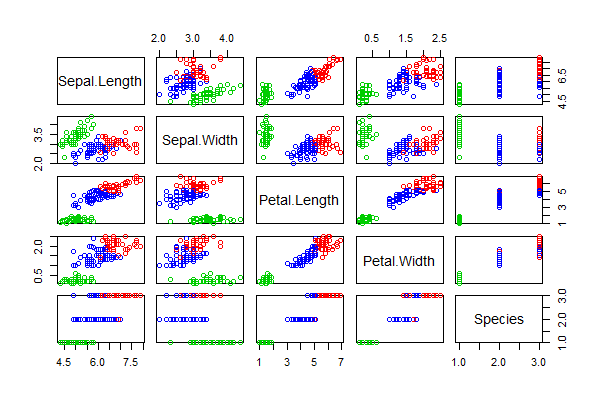``````> library(GGally)
> ggpairs(iris,columns = 1:5,mapping=aes(colour=as.character(res\$cluster)))
``````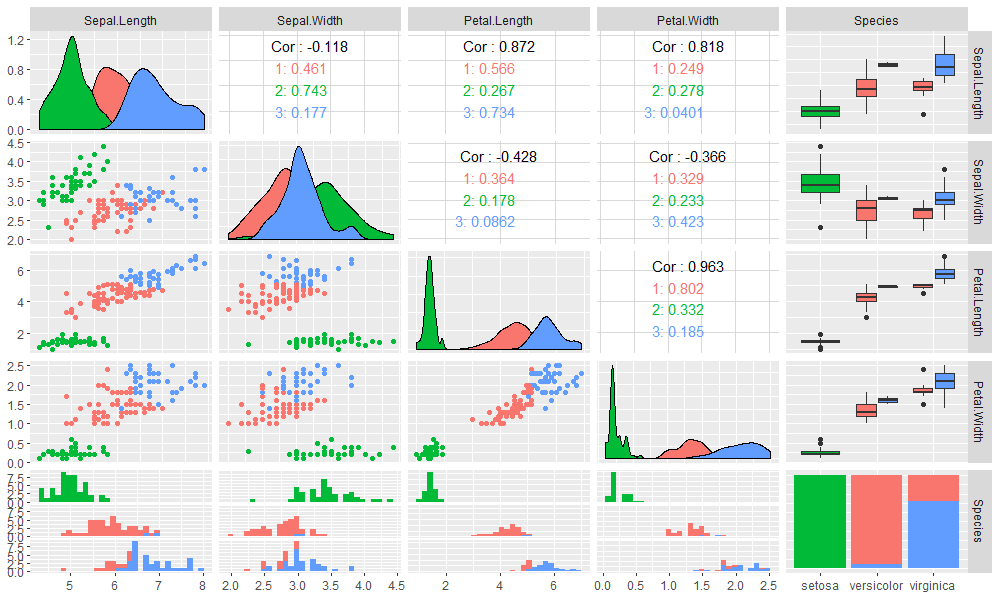``````
> library(flexclust)
> clk2 <- cclust(iris[,-5], k=3);clk2
kcca object of family ‘kmeans’

call:
cclust(x = iris[, -5], k = 3)

cluster sizes:
1  2  3
39 61 50

# 画出段剖面图
> barchart(clk2,legend=TRUE)
``````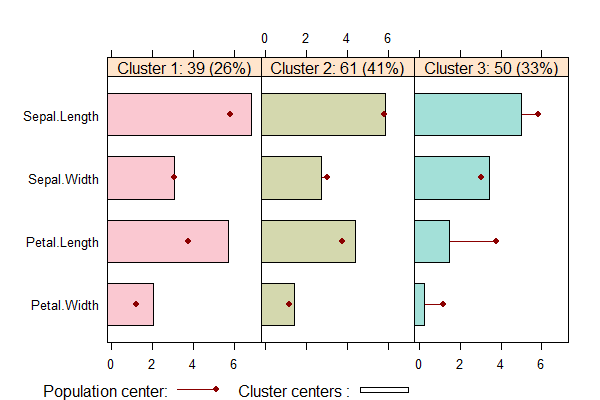• Cluster1中，有39个数据点占26%，Sepal.Width指标在均值附近，其他指标都大于均值。
• Cluster2中，有61个数据点占41%，Sepal.Width指标略小于均值，其他指标在均值附近。
• Cluster3中，有50个数据点占33%，Sepal.Width略大于均值，其他指标都小于均值。